# A theory of transfer learning with applications to active learning

## Abstract

We explore a transfer learning setting, in which a finite sequence of target concepts are sampled independently with an unknown distribution from a known family. We study the total number of labeled examples required to learn all targets to an arbitrary specified expected accuracy, focusing on the asymptotics in the number of tasks and the desired accuracy. Our primary interest is formally understanding the fundamental benefits of transfer learning, compared to learning each target independently from the others. Our approach to the transfer problem is general, in the sense that it can be used with a variety of learning protocols. As a particularly interesting application, we study in detail the benefits of transfer for self-verifying active learning; in this setting, we find that the number of labeled examples required for learning with transfer is often significantly smaller than that required for learning each target independently.

## Introduction

Transfer learning reuses knowledge from past related tasks to ease the process of learning to perform a new task. The goal of transfer learning is to leverage previous learning and experience to more efficiently learn novel, but related, concepts, compared to what would be possible without this prior experience. The utility of transfer learning is typically measured by a reduction in the number of training examples required to achieve a target performance on a sequence of related learning problems, compared to the number required for unrelated problems: i.e., reduced sample complexity. In many real-life scenarios, just a few training examples of a new concept or process is often sufficient for a human learner to grasp the new concept given knowledge of related ones. For example, learning to drive a van becomes much easier a task if we have already learned how to drive a car. Learning French is somewhat easier if we have already learned English (vs. Chinese), and learning Spanish is easier if we know Portuguese (vs. German). We are therefore interested in understanding the conditions that enable a learning machine to leverage abstract knowledge obtained as a by-product of learning past concepts, to improve its performance on future learning problems. Furthermore, we are interested in how the magnitude of these improvements grows as the learning system gains more experience from learning multiple related concepts.

The ability to transfer knowledge gained from previous tasks to make it easier to learn a new task can potentially benefit a wide range of real-world applications, including computer vision, natural language processing, cognitive science (e.g., fMRI brain state classification), and speech recognition, to name a few. As an example, consider training a speech recognizer. After training on a number of individuals, a learning system can identify common patterns of speech, such as accents or dialects, each of which requires a slightly different speech recognizer; then, given a new person to train a recognizer for, it can quickly determine the particular dialect from only a few well-chosen examples, and use the previously-learned recognizer for that particular dialect. In this case, we can think of the transferred knowledge as consisting of the common aspects of each recognizer variant and more generally the distribution of speech patterns existing in the population these subjects are from. This same type of distribution-related knowledge transfer can be helpful in a host of applications, including all those mentioned above.

Supposing these target concepts (e.g., speech patterns) are sampled independently from a fixed population, having knowledge of the distribution of concepts in the population may often be quite valuable. More generally, we may consider a general scenario in which the target concepts are sampled i.i.d. according to a fixed distribution. As we show below, the number of labeled examples required to learn a target concept sampled according to this distribution may be dramatically reduced if we have direct knowledge of the distribution. However, since in many real-world learning scenarios, we do not have direct access to this distribution, it is desirable to be able to somehow learn the distribution, based on observations from a sequence of learning problems with target concepts sampled according to that distribution. The hope is that an estimate of the distribution so-obtained might be almost as useful as direct access to the true distribution in reducing the number of labeled examples required to learn subsequent target concepts. The focus of this paper is an approach to transfer learning based on estimating the distribution of the target concepts. Whereas we acknowledge that there are other important challenges in transfer learning, such as exploring improvements obtainable from transfer under various alternative notions of task relatedness (Evgeniou and Pontil 2004; Ben-David and Schuller 2003), or alternative reuses of knowledge obtained from previous tasks (Thrun 1996), we believe that learning the distribution of target concepts is a central and crucial component in many transfer learning scenarios, and can reduce the total sample complexity across tasks.

Note that it is not immediately obvious that the distribution of targets can even be learned in this context, since we do not have direct access to the target concepts sampled according to it, but rather have only indirect access via a finite number of labeled examples for each task; a significant part of the present work focuses on establishing that as long as these finite labeled samples are larger than a certain size, they hold sufficient information about the distribution over concepts for estimation to be possible. In particular, in contrast to standard results on consistent density estimation, our estimators are not directly based on the target concepts, but rather are only indirectly dependent on these via the labels of a finite number of data points from each task. One desideratum we pay particular attention to is minimizing the number of extra labeled examples needed for each task, beyond what is needed for learning that particular target, so that the benefits of transfer learning are obtained almost as a by-product of learning the targets. Our technique is general, in that it applies to any concept space with finite VC dimension; also, the process of learning the target concepts is (in some sense) decoupled from the mechanism of learning the concept distribution, so that we may apply our technique to a variety of learning protocols, including passive supervised learning, active supervised learning, semi-supervised learning, and learning with certain general data-dependent forms of interaction (Hanneke 2009). For simplicity, we choose to formulate our transfer learning algorithms in the language of active learning; as we show, this problem can benefit significantly from transfer. Formulations for other learning protocols would follow along similar lines, with analogous theorems; only the results in Sect. 5 are specific to active learning.

Transfer learning is related at least in spirit to much earlier work on case-based and analogical learning (Carbonell 1983, 1986; Veloso and Carbonell 1993; Kolodner 1993; Thrun 1996), although that body of work predated modern machine learning, and focused on symbolic reuse of past problem solving solutions rather than on current machine learning problems such as classification, regression or structured learning. More recently, transfer learning (and the closely related problem of multitask learning) has been studied in specific cases with interesting (though sometimes heuristic) approaches (Caruana 1997; Silver 2000; Micchelli and Pontil 2004; Baxter 1997; Ben-David and Schuller 2003). This paper considers a general theoretical framework for transfer learning, based on an Empirical Bayes perspective, and derives rigorous theoretical results on the benefits of transfer. We discuss the relation of this analysis to existing theoretical work on transfer learning below.

### Active learning

Active learning is a powerful form of supervised machine learning characterized by interaction between the learning algorithm and data source during the learning process (Cohn et al. 1994; McCallum and Nigam 1998; Campbell et al. 2000; Tong and Koller 2001; Nguyen and Smeulders 2004; Baram et al. 2004; Donmez et al. 2007; Donmez and Carbonell 2008; He and Carbonell 2008). In this work, we consider a variant known as pool-based active learning, in which a learning algorithm is given access to a (typically very large) collection of unlabeled examples, and is able to select any of those examples, request the supervisor to label it (in agreement with the target concept), then after receiving the label, select another example from the pool, etc. This sequential label-requesting process continues until some halting criterion is reached, at which point the algorithm outputs a classifier, and the objective is for this classifier to closely approximate the (unknown) target concept in the future. The primary motivation behind pool-based active learning is that, often, unlabeled examples are inexpensive and available in abundance, while annotating those examples can be costly or time-consuming; as such, we often wish to select only the informative examples to be labeled, thus reducing information-redundancy to some extent, compared to the baseline of selecting the examples to be labeled uniformly at random from the pool (passive learning).

There has recently been an explosion of fascinating theoretical results on the advantages of this type of active learning, compared to passive learning, in terms of the number of labels required to obtain a prescribed accuracy (called the sample complexity): e.g., Freund et al. (1997), Dasgupta (2004, 2005), Dasgupta et al. (2008, 2009), Hanneke (2007a, 2007b, 2009, 2011), Balcan et al., (2007, 2009, 2010), Wang (2009), Kääriäinen (2006), Friedman (2009), Castro and Nowak (2008), Nowak (2008), Koltchinskii (2010), Beygelzimer et al. (2009). In particular, Balcan et al. (2010) show that in noise-free binary classifier learning, for any passive learning algorithm for a concept space of finite VC dimension, there exists an active learning algorithm with asymptotically much smaller sample complexity for any nontrivial target concept. Thus, it appears there are profound advantages to active learning compared to passive learning. In later work, Hanneke (2009) strengthens this result by removing a certain dependence on the underlying distribution of the data in the learning algorithm.

However, the ability to rapidly converge to a good classifier using only a small number of labels is only one desirable quality of a machine learning method, and there are other qualities that may also be important in certain scenarios. In particular, the ability to verify the performance of a learning method is often a crucial part of machine learning applications, as (among other things) it helps us determine whether we have enough data to achieve a desired level of accuracy with the given method. In passive learning, one common practice for this verification is to hold out a random sample of labeled examples as a validation sample to evaluate the trained classifier (e.g., to determine when training is complete). It turns out this technique is not feasible in active learning, since in order to be really useful as an indicator of whether we have seen enough labels to guarantee the desired accuracy, the number of labeled examples in the random validation sample would need to be much larger than the number of labels requested by the active learning algorithm itself, thus (to some extent) canceling the savings obtained by performing active rather than passive learning. Another common practice in passive learning is to examine the training error rate of the returned classifier, which can serve as a reasonable indicator of performance (after adjusting for model complexity). However, again this measure of performance is not necessarily reasonable for active learning, since the set of examples the algorithm requests the labels of is typically distributed very differently from the test examples the classifier will be applied to after training.

This reasoning seems to indicate that performance verification is (at best) a far more subtle issue in active learning than in passive learning. Indeed, Balcan et al. (2010) note that although the number of labels required to achieve good accuracy in active learning is significantly smaller than passive learning, it is sometimes the case that the number of labels required to verify that the accuracy is good is not significantly improved. In particular, this phenomenon can increase significantly the sample complexity of active learning algorithms that adaptively determine how many labels to request before terminating. In short, if we require the algorithm both to learn an accurate concept and to know that its concept is accurate, then the number of labels required by active learning may sometimes not be significantly smaller than the number required by passive learning.

In the present work, we are interested in the question of whether a form of transfer learning can help to bridge this gap, enabling self-verifying active learning algorithms to obtain the same types of dramatic improvements over passive learning as can be achieved by their non-self-verifying counterparts.

### Outline of the paper

The remainder of the paper is organized as follows. In Sect. 2 we introduce basic notation used throughout, and survey some related work from the existing literature. In Sect. 3, we describe and analyze our proposed method for estimating the distribution of target concepts, the key ingredient in our approach to transfer learning, which we then present in Sect. 4. Finally, in Sect. 5, we investigate the benefits of this type of transfer learning for self-verifying active learning.

## Definitions and related work

First, we state a few basic notational conventions. We denote ℕ={1,2,…} and ℕ0=ℕ∪{0}. For any random variable X, we generally denote by ℙ X the distribution of X (the induced probability measure on the range of X), and by ℙ X|Y the regular conditional distribution of X given Y. For any pair of probability measures μ 1,μ 2 on a measurable space $$(\varOmega, \mathcal{F})$$, we define

$$\| \mu_1 - \mu_2 \| = \sup_{A \in\mathcal{F}} \bigl| \mu_1(A) - \mu_2(A) \bigr|.$$

Next we define the particular objects of interest to our present discussion. Let Θ be an arbitrary set (called the parameter space), $$(\mathcal{X},\mathcal{B}_{\mathcal{X}})$$ be a Borel space (Schervish 1995) (where $$\mathcal{X}$$ is called the instance space), and $$\mathcal{D}$$ be a fixed distribution on $$\mathcal{X}$$ (called the data distribution). For instance, Θ could be ℝn and $$\mathcal {X}$$ could be ℝm, for some n,m∈ℕ, though more general scenarios are certainly possible as well, including infinite-dimensional parameter spaces. Let ℂ be a set of measurable classifiers $$h : \mathcal {X}\to\{-1,+1\}$$ (called the concept space), and suppose ℂ has VC dimension d<∞ (Vapnik 1982) (such a space is called a VC class). ℂ is equipped with its Borel σ-algebra $$\mathcal{B}$$, induced by the pseudo-metric $$\rho(h,g) = \mathcal{D}(\{x \in\mathcal{X}: h(x) \neq g(x)\})$$. Though all of our results can be formulated for general $$\mathcal{D}$$ in slightly more complex terms, for simplicity throughout the discussion below we suppose ρ is actually a metric, in that any h,g∈ℂ with hg have ρ(h,g)>0; this amounts to a topological assumption on ℂ relative to $$\mathcal{D}$$.

For each θΘ, π θ is a distribution on ℂ (called a prior). Our only (rather mild) assumption on this family of prior distributions is that {π θ :θΘ} be totally bounded, in the sense that ∀ε>0, ∃ finite Θ ε Θ s.t. ∀θΘ,∃θ ε Θ ε with $$\|\pi_{\theta} - \pi_{\theta _{\varepsilon}}\| < \varepsilon$$. See Devroye and Lugosi (2001) for examples of categories of classes that satisfy this.

The general setup for the learning problem is that we have a true parameter value θ Θ, and a collection of ℂ-valued random variables $$\{ h^{*}_{t\theta}\}_{t \in\mathbb{N}, \theta\in\varTheta}$$, where for a fixed θΘ the $$\{h^{*}_{t\theta}\}_{t \in\mathbb{N}}$$ variables are i.i.d. with distribution π θ .

The learning problem is the following. For each θΘ, there is a sequence

$$\mathcal{Z}_{t}(\theta) = \bigl\{\bigl(X_{t1},Y_{t1}( \theta )\bigr),\bigl(X_{t2},Y_{t2}(\theta)\bigr),\ldots\bigr \},$$

where {X ti } t,i∈ℕ are i.i.d. $$\mathcal{D}$$, and for each t,i∈ℕ, $$Y_{ti}(\theta) = h^{*}_{t\theta}(X_{ti})$$. For k∈ℕ we denote by $$\mathcal{Z}_{tk}(\theta) = \{ (X_{t1},Y_{t1}(\theta)),\ldots,(X_{tk},Y_{tk}(\theta))\}$$. Since the Y ti (θ) are the actual $$h^{*}_{t\theta}(X_{ti})$$ values, we are studying the non-noisy, or realizable-case, setting.

The algorithm receives values ε and T as input, and for each t∈{1,2,…,T} in increasing order, it observes the sequence X t1,X t2,… , and may then select an index i 1, receive label $$Y_{ti_{1}}({\theta_{\star}})$$, select another index i 2, receive label $$Y_{ti_{2}}({\theta_{\star }})$$, etc. The algorithm proceeds in this fashion, sequentially requesting labels, until eventually it produces a classifier $$\hat{h}_{t}$$. It then increments t and repeats this process until it produces a sequence $$\hat{h}_{1},\hat{h}_{2},\ldots,\hat{h}_{T}$$, at which time it halts. To be called correct, the algorithm must have a guarantee that $$\forall{\theta_{\star}}\in\varTheta, \forall t \leq T, \mathbb {E} [\rho (\hat{h}_{t},h^{*}_{t{\theta_{\star}}} ) ] \leq\varepsilon$$, for any values of T∈ℕ and ε>0 given as input. We will be interested in the expected number of label requests necessary for a correct learning algorithm, averaged over the T tasks, and in particular in how shared information between tasks can help to reduce this quantity when direct access to θ is not available to the algorithm.

### Relation to existing theoretical work on transfer learning

Although we know of no existing work on the theoretical advantages of transfer learning for active learning, the existing literature contains several analyses of the advantages of transfer learning for passive learning. In his classic work, Baxter (1997, Sect. 4) explores a similar setup for a general form of passive learning, except in a full Bayesian setting (in contrast to our setting, often referred to as “empirical Bayes,” which includes a constant parameter θ to be estimated from data). Essentially, Baxter (1997) sets up a hierarchical Bayesian model, in which (in our notation) θ is a random variable with known distribution (hyper-prior), but otherwise the specialization of Baxter’s setting to the pattern recognition problem is essentially identical to our setup above. This hyper-prior does make the problem slightly easier, but generally the results of Baxter (1997) are of a different nature than our objectives here. Specifically, Baxter’s results on learning from labeled examples can be interpreted as indicating that transfer learning can improve certain constant factors in the asymptotic rate of convergence of the average of expected error rates across the learning problems. That is, certain constant complexity terms (for instance, related to the concept space) can be reduced to (potentially much smaller) values related to $$\pi_{{\theta _{\star}}}$$ by transfer learning. Baxter argues that, as the number of tasks grows large, this effectively achieves close to the known results on the sample complexity of passive learning with direct access to θ . A similar claim is discussed by Ando and Zhang (2004) (though in less detail) for a setting closer to that studied here, where θ is an unknown parameter to be estimated.

There are also several results on transfer learning of a slightly different variety, in which, rather than having a prior distribution for the target concept, the learner initially has several potential concept spaces to choose from, and the role of transfer is to help the learner select from among these concept spaces (Baxter 2000; Ando and Zhang 2005). In this case, the idea is that one of these concept spaces has the best average minimum achievable error rate per learning problem, and the objective of transfer learning is to perform nearly as well as if we knew which of the spaces has this property. In particular, if we assume the target functions for each task all reside in one of the concept spaces, then the objective of transfer learning is to perform nearly as well as if we knew which of the spaces contains the targets. Thus, transfer learning results in a sample complexity related to the number of learning problems, a complexity term for this best concept space, and a complexity term related to the diversity of concept spaces we have to choose from. In particular, as with Baxter (1997), these results can typically be interpreted as giving constant factor improvements from transfer in a passive learning context, at best reducing the complexity constants, from those for the union over the given concept spaces, down to the complexity constants of the single best concept space.

In addition to the above works, there are several analyses of transfer learning and multitask learning of an entirely different nature than our present discussion, in that the objectives of the analysis are somewhat different. Specifically, there is a branch of the literature concerned with task relatedness, not in terms of the underlying process that generates the target concepts, but rather directly in terms of relations between the target concepts themselves. In this sense, several tasks with related target concepts should be much easier to learn than tasks with unrelated target concepts. This is studied in the context of kernel methods by Micchelli and Pontil (2004), Evgeniou and Pontil (2004), Evgeniou et al. (2005), and in a more general theoretical framework by Ben-David and Schuller (2003). As mentioned, our approach to transfer learning is based on the idea of estimating the distribution of target concepts. As such, though interesting and important, these notions of direct relatedness of target concepts are not as relevant to our present discussion.

As with Baxter (1997), the present work is interested in showing that as the number of tasks grows large, we can effectively achieve a sample complexity close to that achievable with direct access to θ . However, in contrast, we are interested in a general approach to transfer learning and the analysis thereof, leading to concrete results for a variety of learning protocols such as active learning and semi-supervised learning. In particular, our analysis of active learning reveals the interesting phenomenon that transfer learning can sometimes improve the asymptotic dependence on ε, rather than merely the constant factors as in the analysis of Baxter (1997).

Our work contrasts with Baxter (1997) in another important respect, which significantly changes the way we approach the problem. Specifically, in Baxter’s analysis, the results (e.g., Baxter 1997, Theorems 4, 6) regard the average loss over the tasks, and are stated as a function of the number of samples per task. This number of samples plays a dual role in Baxter’s analysis, since these samples are used both by the individual learning algorithm for each task, and also for the global transfer learning process that provides the learners with information about θ . Baxter is then naturally interested in the rates at which these losses shrink as the sample sizes grow large, and therefore formulates the results in terms of the asymptotic behavior as the per-task sample sizes grow large. In particular, the results of Baxter (1997) involve residual terms which become negligible for large sample sizes, but may be more significant for smaller sample sizes.

In our work, we are interested in decoupling these two roles for the sample sizes; in particular, our results regard only the number of tasks as an asymptotic variable, while the number of samples per task remains bounded. First, we note a very practical motivation for this: namely, non-altruistic learners. In many settings where transfer learning may be useful, it is desirable that the number of labeled examples we need to collect from each particular learning problem never be significantly larger than the number of such examples required to solve that particular problem (i.e., to learn that target concept to the desired accuracy). For instance, this is the case when the learning problems are not all solved by the same individual (or company, etc.), but rather a coalition of cooperating individuals (e.g., hospitals sharing data on clinical trials); each individual may be willing to share the data they used to learn their particular concept, in the interest of making others’ learning problems easier; however, they may not be willing to collect significantly more data than they themselves need for their own learning problem. We should therefore be particularly interested in studying transfer as a by-product of the usual learning process; failing this, we are interested in the minimum possible number of extra labeled examples per task to gain the benefits of transfer learning.

The issue of non-altruistic learners also presents a further technical problem in that the individuals solving each task may be unwilling to alter their method of gathering data to be more informative for the transfer learning process. That is, we expect the learning process for each task is designed with the sole intention of estimating the target concept, without regard for the global transfer learning problem. To account for this, we model the transfer learning problem in a reduction-style framework, in which we suppose there is some black-box learning algorithm to be run for each task, which takes a prior as input and has a theoretical guarantee of good performance provided the prior is correct. We place almost no restrictions whatsoever on this learning algorithm, including the manner in which it accesses the data. This allows remarkable generality, since this procedure could be passive, active, semi-supervised, or some other kind of query-based strategy. However, because of this generality, we have no guarantee on the information about θ reflected in the data used by this algorithm (especially if it is an active learning algorithm). As such, we choose not to use the label information gathered by the learning algorithm for each task when estimating the θ , but instead take a small number of additional random labeled examples from each task with which to estimate θ . Again, we want to minimize this number of additional samples per task; indeed, in this work we are able to make due with a mere constant number of additional samples per task. To our knowledge, no result of this type (estimating θ using a bounded sample size per learning problem) has previously been established at the level of generality studied here.

## Estimating the prior

The advantage of transfer learning in this setting is that each learning problem provides some information about θ , so that after solving several of the learning problems, we might hope to be able to estimate θ . Then, with this estimate in hand, we can use the corresponding estimated prior distribution in the learning algorithm for subsequent learning problems, to help inform the learning process similarly to how direct knowledge of θ might be helpful. However, the difficulty in approaching this is how to define such an estimator. Since we do not have direct access to the $$h^{*}_{t}$$ values, but rather only indirect observations via a finite number of example labels, the standard results for density estimation from i.i.d. samples cannot be applied.

The idea we pursue below is to consider the distributions on $$\mathcal {Z}_{t k}({\theta_{\star}})$$. These variables are directly observable, by requesting the labels of those examples. Thus, for any finite k∈ℕ, this distribution is estimable from observable data. That is, using the i.i.d. values $$\mathcal{Z}_{1 k}({\theta_{\star }}), \ldots, \mathcal{Z}_{t k}({\theta_{\star}})$$, we can apply standard techniques for density estimation to arrive at an estimator of $$\mathbb{P}_{\mathcal{Z}_{t k}({\theta_{\star}})}$$. Then the question is whether the distribution $$\mathbb{P}_{\mathcal{Z}_{t k}({\theta_{\star}})}$$ uniquely characterizes the prior distribution $$\pi_{{\theta_{\star}}}$$: that is, whether $$\pi_{{\theta_{\star}}}$$ is identifiable from $$\mathbb{P}_{\mathcal{Z}_{t k}({\theta_{\star}})}$$.

As an example, consider the space of half-open interval classifiers on [0,1]: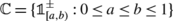, where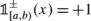if ax<b and −1 otherwise. In this case, $$\pi_{{\theta_{\star}}}$$ is not necessarily identifiable from $$\mathbb{P}_{\mathcal{Z}_{t 1}({\theta_{\star }})}$$; for instance, the distributions $$\pi_{\theta_{1}}$$ and $$\pi_{\theta_{2}}$$ characterized by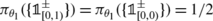and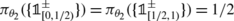are not distinguished by these one-dimensional distributions. However, it turns out that for this half-open intervals problem, $$\pi_{{\theta_{\star}}}$$ is uniquely identifiable from $$\mathbb{P}_{\mathcal{Z}_{t 2}({\theta_{\star}})}$$; for instance, in the θ 1 vs. θ 2 scenario, the conditional probability $$\mathbb{P}_{(Y_{t 1}(\theta_{i}),Y_{t 2}(\theta _{i})) | (X_{t 1},X_{t 2})}( (+1,+1) | (1/4,3/4))$$ will distinguish $$\pi_{\theta_{1}}$$ from $$\pi_{\theta_{2}}$$, and this can be calculated from $$\mathbb{P}_{\mathcal{Z}_{t 2}(\theta_{i})}$$. The crucial element of the analysis below is determining the appropriate value of k to uniquely identify $$\pi_{{\theta_{\star}}}$$ from $$\mathbb {P}_{\mathcal {Z}_{t k}({\theta_{\star}})}$$ in general. As we will see, k=d (the VC dimension) is always sufficient, a key insight for the results that follow. We will also see this is not the case for any k<d.

To be specific, in order to transfer knowledge from one task to the next, we use a few labeled data points from each task to gain information about θ . For this, for each task t, we simply take the first d data points in the $$\mathcal{Z}_{t}({\theta_{\star}})$$ sequence. That is, we request the labels

$$Y_{t1}({\theta_{\star}}),Y_{t2}({ \theta_{\star}}),\ldots ,Y_{td}({\theta_{\star}})$$

and use the points $$\mathcal{Z}_{t d}({\theta_{\star}})$$ to update an estimate of θ .

The following result shows that this technique does provide a consistent estimator of $$\pi_{{\theta_{\star}}}$$. Again, note that this result is not a straightforward application of the standard approach to consistent estimation, since the observations here are not the $$h^{*}_{t {\theta_{\star}}}$$ variables themselves, but rather a number of the Y ti (θ ) values. The key insight in this result is that $$\pi_{{\theta_{\star}}}$$ is uniquely identified by the joint distribution $$\mathbb{P}_{\mathcal{Z}_{t d}({\theta_{\star}})}$$ over the first d labeled examples; later, we prove this is not necessarily true for $$\mathbb{P}_{\mathcal{Z}_{t k}({\theta_{\star}})}$$ for values k<d. This identifiability result is stated below in Corollary 1; as we discuss in Sect. 3.1, there is a fairly simple direct proof of this result. However, for our purposes, we will actually require the stronger condition that any θΘ with small $$\|\mathbb{P}_{\mathcal{Z}_{t k}(\theta)} - \mathbb{P}_{\mathcal{Z}_{t k}({\theta_{\star}})}\|$$ also has small $$\|\pi_{\theta} - \pi_{{\theta_{\star}}}\|$$. This stronger requirement adds to the complexity of the proofs. The results in this section are purely concerned with relating distances in the space of $$\mathbb{P}_{\mathcal{Z}_{t d}(\theta)}$$ distributions to the corresponding distances in the space of π θ distributions; as such, they are not specific to active learning or other learning protocols, and hence are of independent interest.

### Theorem 1

There exists an estimator $$\hat{\theta}_{T {\theta_{\star}}} = \hat {\theta}_{T}(\mathcal{Z}_{1d}({\theta_{\star}}), \ldots, \mathcal {Z}_{Td}({\theta_{\star}}))$$, and functions R:ℕ0×(0,1]→[0,∞) and δ:ℕ0×(0,1]→[0,1], such that for any α>0, lim T→∞ R(T,α)=lim T→∞ δ(T,α)=0 and for any T∈ℕ0 and θ Θ,

$$\mathbb{P} \bigl( \| \pi_{\hat{\theta}_{T {\theta_{\star}}}} - \pi_{{\theta_{\star}}} \| > R(T,\alpha) \bigr) \leq\delta(T,\alpha) \leq\alpha.$$

One important detail to note, for our purposes, is that R(T,α) is independent from θ , so that the value of R(T,α) can be calculated and used within a learning algorithm. The proof of Theorem 1 will be established via the following sequence of lemmas. Lemma 1 relates distances in the space of priors to distances in the space of distributions on the full data sets. In turn, Lemma 2 relates these distances to distances in the space of distributions on a finite number of examples from the data sets. Lemma 3 then relates the distances between distributions on any finite number of examples to distances between distributions on d examples. Finally, Lemma 4 presents a standard result on the existence of a converging estimator, in this case for the distribution on d examples, for totally bounded families of distributions. Tracing these relations back, they relate convergence of the estimator for the distribution of d examples to convergence of the corresponding estimator for the prior itself.

### Lemma 1

For any θ,θ′∈Θ and t∈ℕ,

$$\|\pi_{\theta} - \pi_{\theta^{\prime}}\| = \| \mathbb{P}_{\mathcal{Z}_{t}(\theta)} - \mathbb{P}_{\mathcal {Z}_{t}(\theta ^{\prime})}\|.$$

### Proof

Fix θ,θ′∈Θ, t∈ℕ. Let $$\mathbb{X}= \{X_{t1},X_{t2},\ldots\}$$, $$\mathbb{Y}(\theta) = \{ Y_{t1}(\theta), Y_{t2}(\theta), \ldots\}$$, and for k∈ℕ let $$\mathbb{X}_{k} = \{X_{t1},\ldots ,X_{tk}\}$$. and $$\mathbb{Y}_{k}(\theta) = \{Y_{t1}(\theta),\ldots,Y_{tk}(\theta )\}$$. For h∈ℂ, let $$c_{\mathbb{X}}(h) = \{ (X_{t1},h(X_{t1})),(X_{t2},h(X_{t2})), \ldots\}$$.

For h,g∈ℂ, define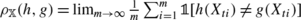(if the limit exists), and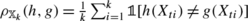. Note that since ℂ has finite VC dimension, so does the collection of sets {{x:h(x)≠g(x)}:h,g∈ℂ}, so that the uniform strong law of large numbers implies that with probability one, ∀h,g∈ℂ, $$\rho_{\mathbb{X}}(h,g)$$ exists and has $$\rho_{\mathbb{X}}(h,g) = \rho(h,g)$$ (Vapnik 1982).

Consider any θ,θ′∈Θ, and any $$A \in \mathcal{B}$$. Then since $$\mathcal{B}$$ is the Borel σ-algebra induced by ρ, any hA has ∀gA, ρ(h,g)>0. Thus, if $$\rho_{\mathbb{X}}(h,g) = \rho(h,g)$$ for all h,g∈ℂ, then ∀hA,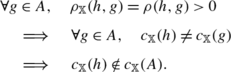This implies $$c_{\mathbb{X}}^{-1}(c_{\mathbb{X}}(A)) = A$$. Under these conditions,

$$\mathbb{P}_{\mathcal{Z}_{t}(\theta) | \mathbb{X}}\bigl(c_{\mathbb {X}}(A)\bigr) = \pi_{\theta} \bigl(c_{\mathbb{X}}^{-1}\bigl(c_{\mathbb{X}}(A)\bigr)\bigr) = \pi_{\theta}(A),$$

and similarly for θ′.

Any measurable set C for the range of $$\mathcal{Z}_{t}(\theta)$$ can be expressed as $$C = \{c_{\bar{x}}(h) : (h, \bar{x}) \in C^{\prime}\}$$ for some appropriate $$C^{\prime} \in\mathcal{B}\otimes\mathcal{B}_{\mathcal{X} }^{\infty}$$. Letting $$C^{\prime}_{\bar{x}} = \{ h : (h,\bar{x}) \in C^{\prime}\}$$, we have

$$\mathbb{P}_{\mathcal{Z}_{t}(\theta)}(C) = \int\pi_{\theta}\bigl(c_{\bar{x}}^{-1} \bigl(c_{\bar{x}}\bigl(C^{\prime}_{\bar {x}}\bigr)\bigr)\bigr) \mathbb{P}_{\mathbb{X}}\, (\mathrm{d}\bar{x}) = \int\pi_{\theta} \bigl(C^{\prime}_{\bar{x}}\bigr) \mathbb{P}_{\mathbb {X}}\,(\mathrm{d} \bar{x}) = \mathbb{P}_{(h^{*}_{t \theta},\mathbb{X})}\bigl(C^{\prime}\bigr).$$

Likewise, this reasoning holds for θ′. Then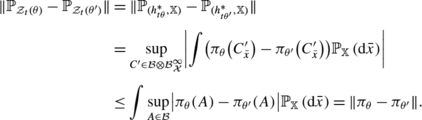Since $$h^{*}_{t \theta}$$ and $$\mathbb{X}$$ are independent, for $$A \in \mathcal{B}$$, $$\pi_{\theta}(A) = \mathbb{P}_{h^{*}_{t \theta}}(A) = \mathbb {P}_{h^{*}_{t \theta }}(A) \mathbb{P}_{\mathbb{X}}(\mathcal{X}^{\infty}) = \mathbb {P}_{(h^{*}_{t \theta },\mathbb{X})}(A \times\mathcal{X}^{\infty})$$. Analogous reasoning holds for $$h^{*}_{t \theta^{\prime}}$$. Thus, we have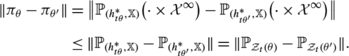Combining the above, we have $$\| \mathbb{P}_{\mathcal{Z}_{t}(\theta )} - \mathbb{P}_{\mathcal{Z}_{t}(\theta^{\prime})} \| = \|\pi_{\theta} - \pi_{\theta^{\prime}}\|$$. □

### Lemma 2

There exists a sequence r k =o(1) such thatt,k∈ℕ, ∀θ,θ′∈Θ,

$$\| \mathbb{P}_{\mathcal{Z}_{t k}(\theta)} - \mathbb{P}_{\mathcal {Z}_{t k}(\theta ^{\prime})} \| \leq\| \pi_{\theta} - \pi_{\theta^{\prime}} \| \leq\| \mathbb{P}_{\mathcal{Z}_{t k}(\theta)} - \mathbb {P}_{\mathcal{Z}_{t k}(\theta^{\prime})} \| + r_{k}.$$

### Proof

The left inequality follows from Lemma 1 and the basic definition of ∥⋅∥, since $$\mathbb{P}_{\mathcal{Z}_{t k}(\theta)}(\cdot) = \mathbb {P}_{\mathcal {Z}_{t}(\theta)}(\cdot\times(\mathcal{X}\times\{-1,+1\})^{\infty})$$, so that

$$\| \mathbb{P}_{\mathcal{Z}_{t k}(\theta)} - \mathbb{P}_{\mathcal {Z}_{t k}(\theta ^{\prime})} \| \leq\| \mathbb{P}_{\mathcal{Z}_{t}(\theta)} - \mathbb{P}_{\mathcal {Z}_{t}(\theta ^{\prime})} \| = \| \pi_{\theta} - \pi_{\theta^{\prime}} \|.$$

The remainder of this proof focuses on the right inequality. Fix θ,θ′∈Θ, let γ>0, and let $$B \subseteq(\mathcal{X}\times\{-1,+1\})^{\infty}$$ be a measurable set such that

$$\| \pi_{\theta} - \pi_{\theta^{\prime}} \| = \| \mathbb {P}_{\mathcal {Z}_{t}(\theta)} - \mathbb{P}_{\mathcal{Z}_{t}(\theta^{\prime})} \| < \mathbb{P}_{\mathcal{Z}_{t}(\theta)}(B) - \mathbb{P}_{\mathcal{Z}_{t}(\theta ^{\prime })}(B) + \gamma.$$

Let $$\mathcal {A}$$ be the collection of all measurable subsets of $$(\mathcal{X} \times\{-1,+1\})^{\infty}$$ representable in the form $$A^{\prime} \times(\mathcal{X}\times\{ -1,+1\} )^{\infty}$$, for some measurable $$A^{\prime} \subseteq(\mathcal{X}\times\{-1,+1\})^{k}$$ and some k∈ℕ. In particular, since $$\mathcal {A}$$ is an algebra that generates the product σ-algebra, Carathéodory’s extension theorem (Schervish 1995) implies that there exist disjoint sets {A i } i∈ℕ in $$\mathcal {A}$$ such that B⊆⋃ i∈ℕ A i and

$$\mathbb{P}_{\mathcal{Z}_{t}(\theta)}(B) - \mathbb{P}_{\mathcal {Z}_{t}(\theta ^{\prime})}(B) < \sum _{i \in\mathbb{N}} \mathbb{P}_{\mathcal{Z}_{t}(\theta )}(A_i) - \sum _{i \in\mathbb{N}} \mathbb{P}_{\mathcal{Z}_{t}(\theta^{\prime })}(A_i) + \gamma.$$

Additionally, as these sums are bounded, there must exist n∈ℕ such that

$$\sum_{i \in\mathbb{N}} \mathbb{P}_{\mathcal{Z}_{t}(\theta)}(A_i) < \gamma + \sum_{i = 1}^{n} \mathbb{P}_{\mathcal{Z}_{t}(\theta)}(A_i),$$

so that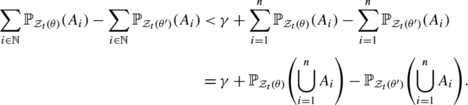As $$\bigcup_{i=1}^{n} A_{i} \in \mathcal {A}$$, there exists k′∈ℕ and measurable $$A^{\prime} \subseteq(\mathcal{X}\times\{-1,+1\})^{k^{\prime}}$$ such that $$\bigcup_{i=1}^{n} A_{i} = A^{\prime} \times(\mathcal{X}\times\{ -1,+1\} )^{\infty}$$, and therefore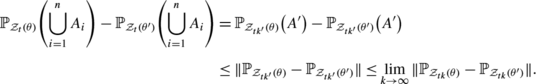In summary, we have $$\| \pi_{\theta} - \pi_{\theta^{\prime}}\| \leq\lim_{k \to\infty} \| \mathbb{P}_{\mathcal{Z}_{t k}(\theta)} - \mathbb{P}_{\mathcal{Z}_{t k}(\theta^{\prime})} \| + 3\gamma$$. Since this is true for an arbitrary γ>0, taking the limit as γ→0 implies

$$\| \pi_{\theta} - \pi_{\theta^{\prime}}\| \leq\lim_{k \to\infty}\| \mathbb{P}_{\mathcal{Z}_{t k}(\theta)} - \mathbb{P}_{\mathcal{Z}_{t k}(\theta^{\prime})} \|.$$

In particular, this implies there exists a sequence r k (θ,θ′)=o(1) such that

$$\forall k \in\mathbb{N},\quad \| \pi_{\theta} - \pi_{\theta^{\prime}}\| \leq\| \mathbb{P}_{\mathcal{Z}_{t k}(\theta)} - \mathbb {P}_{\mathcal{Z}_{t k}(\theta^{\prime})} \| + r_{k} \bigl(\theta,\theta^{\prime}\bigr).$$

This would suffice to establish the upper bound if we were allowing r k to depend on the particular θ and θ′. However, to guarantee the same rates of convergence for all pairs of parameters requires an additional argument. Specifically, let γ>0 and let Θ γ denote a minimal subset of Θ such that, ∀θΘ, ∃θ γ Θ γ s.t. $$\| \pi_{\theta} - \pi_{\theta_{\gamma}} \| < \gamma$$: that is, a minimal γ-cover. Since |Θ γ |<∞ by assumption, defining $$r_{k}(\gamma) = \max_{\theta,\theta^{\prime} \in\varTheta _{\gamma}} r_{k}(\theta,\theta^{\prime})$$, we have r k (γ)=o(1). Furthermore, for any θ,θ′∈Θ, letting $$\theta_{\gamma} = \mathop {\mathrm {argmin}}_{\theta^{\prime\prime} \in \varTheta_{\gamma}} \| \pi_{\theta} - \pi_{\theta^{\prime\prime }} \|$$ and $$\theta_{\gamma}^{\prime} = \mathop {\mathrm {argmin}}_{\theta^{\prime\prime} \in\varTheta_{\gamma}} \| \pi_{\theta^{\prime}} - \pi_{\theta ^{\prime\prime}} \|$$, we have (by triangle inequalities)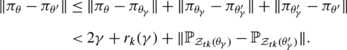By triangle inequalities and the left inequality from the lemma statement (established above), we also have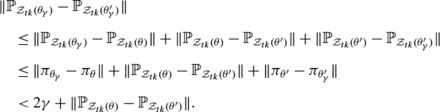Defining r k =inf γ>0(4γ+r k (γ)), we have the right inequality of the lemma statement, and since r k (γ)=o(1) for each γ>0, we have r k =o(1). □

### Lemma 3

t,k∈ℕ, ∀θ,θ′∈Θ,

$$\| \mathbb{P}_{\mathcal{Z}_{t k}(\theta)} - \mathbb{P}_{\mathcal {Z}_{t k}(\theta ^{\prime})} \| \leq4 \cdot2^{2k+d} k^{d} \sqrt{\| \mathbb{P}_{\mathcal{Z}_{t d}(\theta)} - \mathbb{P}_{\mathcal{Z}_{t d}(\theta^{\prime})} \|}.$$

### Proof

Fix any t∈ℕ, and let $$\mathbb{X}= \{X_{t1},X_{t2},\ldots\}$$ and $$\mathbb{Y}(\theta) = \{ Y_{t1}(\theta), Y_{t2}(\theta), \ldots\}$$, and for k∈ℕ let $$\mathbb{X}_{k} = \{X_{t1},\ldots ,X_{tk}\}$$ and $$\mathbb{Y}_{k}(\theta) = \{Y_{t1}(\theta),\ldots,Y_{tk}(\theta )\}$$.

If kd, then $$\mathbb{P}_{\mathcal{Z}_{t k}(\theta)}(\cdot) = \mathbb {P}_{\mathcal{Z}_{t d}(\theta)}(\cdot\times(\mathcal{X}\times\{-1,+1\})^{d-k})$$, so that

$$\|\mathbb{P}_{\mathcal{Z}_{t k}(\theta)} - \mathbb{P}_{\mathcal {Z}_{t k}(\theta ^{\prime})}\| \leq\| \mathbb{P}_{\mathcal{Z}_{t d}(\theta)} - \mathbb{P}_{\mathcal {Z}_{t d}(\theta^{\prime})}\|,$$

and therefore the result trivially holds.

Now suppose k>d. For a sequence $$\bar{z}$$ and I⊆ℕ, we will use the notation $$\bar{z}_{I} = \{\bar{z}_{i} : i \in\nobreak I\}$$. Note that, for any k>d and $$\bar{x}^{k} \in\mathcal{X}^{k}$$, there is a sequence $$\bar{y}(\bar{x}^{k}) \in\{-1,+1\}^{k}$$ such that no h∈ℂ has $$h(\bar{x}^{k}) = \bar{y}(\bar {x}^{k})$$ (i.e., ∀h∈ℂ, ∃ik s.t. $$h(\bar {x}^{k}_{i}) \neq \bar{y}_{i}(\bar{x}^{k})$$). Now suppose k>d and take as an inductive hypothesis that there is a measurable set $$A^{*} \subseteq\mathcal{X}^{\infty}$$ of probability one with the property that $$\forall\bar{x} \in A^{*}$$, for every finite I⊂ℕ with |I|>d, for every $$\bar{y} \in\{-1,+1\}^{\infty}$$ with $$\| \bar{y}_{I} - \bar{y}(\bar{x}_{I})\|_{1}/2 \leq k-1$$,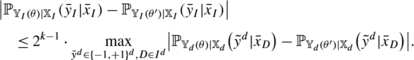This clearly holds for $$\| \bar{y}_{I} - \bar{y}(\bar{x}_{I})\|_{1}/2 = 0$$, since $$\mathbb{P}_{\mathbb{Y}_{I}(\theta) | \mathbb{X}_{I}}(\bar {y}_{I} | \bar{x}_{I}) = 0$$ in this case, so this will serve as our base case in the inductive proof. Next we inductively extend this to the value k>0. Specifically, let $$A^{*}_{k-1}$$ be the A guaranteed to exist by the inductive hypothesis, and fix any $$\bar{x} \in A^{*}$$, $$\bar{y} \in\{-1,+1\}^{\infty}$$, and finite I⊂ℕ with |I|>d and $$\|\bar{y}_{I} - \bar{y}(\bar{x}_{I})\|_{1}/2 = k$$. Let iI be such that $$\bar{y}_{i} \neq\bar{y}_{i}(\bar{x}_{I})$$, and let $$\bar {y}^{\prime} \in\{-1,+1\}$$ have $$\bar{y}^{\prime}_{j} = \bar{y}_{j}$$ for every ji, and $$\bar{y}^{\prime}_{i} = - \bar{y}_{i}$$. Then

$$\mathbb{P}_{\mathbb{Y}_{I}(\theta) | \mathbb{X}_{I}}(\bar {y}_{I}| \bar{x}_{I}) = \mathbb{P}_{\mathbb{Y}_{I\setminus\{i\}}(\theta) | \mathbb {X}_{I\setminus\{i\}}}(\bar{y}_{I\setminus\{i\}}|\bar {x}_{I\setminus\{i\}}) - \mathbb{P}_{\mathbb{Y}_{I}(\theta) | \mathbb{X}_{I}}\bigl(\bar {y}^{\prime }_{I}| \bar{x}_{I}\bigr),$$
(1)

and similarly for θ′. By the inductive hypothesis, this means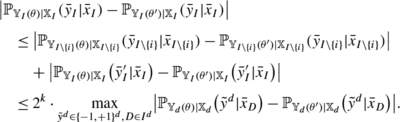Therefore, by the principle of induction, this inequality holds for all k>d, for every $$\bar{x} \in A^{*}$$, $$\bar{y} \in\{-1,+1\}^{\infty}$$, and finite I⊂ℕ, where A has $$\mathcal{D}^{\infty}$$-probability one.

In particular, we have that for θ,θ′∈Θ,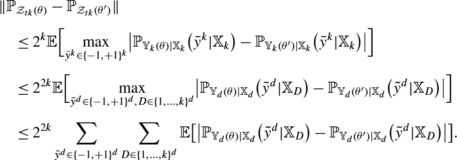Exchangeability implies this is at most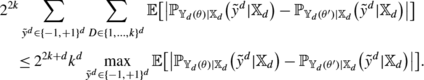To complete the proof, we need only bound this value by an appropriate function of $$\|\mathbb{P}_{\mathcal{Z}_{t d}(\theta)}-\mathbb{P}_{\mathcal {Z}_{t d}(\theta ^{\prime})}\|$$. Toward this end, suppose

$$\mathbb{E} \bigl[ \bigl \vert \mathbb{P}_{\mathbb{Y}_{d}(\theta) | \mathbb {X}_{d}}\bigl( \tilde{y}^{d} | \mathbb{X}_{d}\bigr) - \mathbb{P}_{\mathbb {Y}_{d}(\theta^{\prime})|\mathbb{X}_{d}} \bigl(\tilde{y}^{d} | \mathbb {X}_{d}\bigr)\bigr \vert \bigr] \geq\varepsilon,$$

for some $$\tilde{y}^{d}$$. Then either

$$\mathbb{P} \bigl( \mathbb{P}_{\mathbb{Y}_{d}(\theta) | \mathbb {X}_{d}}\bigl(\tilde {y}^{d} | \mathbb{X}_{d}\bigr) - \mathbb{P}_{\mathbb{Y}_{d}(\theta ^{\prime })|\mathbb{X}_{d}}\bigl( \tilde{y}^{d} | \mathbb{X}_{d}\bigr) \geq\varepsilon / 4 \bigr) \geq\varepsilon/ 4,$$

or

$$\mathbb{P} \bigl( \mathbb{P}_{\mathbb{Y}_{d}(\theta^{\prime}) | \mathbb{X}_{d}}\bigl(\tilde{y}^{d} | \mathbb{X}_{d}\bigr) - \mathbb{P}_{\mathbb{Y}_{d}(\theta)|\mathbb{X}_{d}} \bigl(\tilde{y}^{d} | \mathbb{X}_{d}\bigr) \geq\varepsilon/ 4 \bigr) \geq\varepsilon/ 4.$$

For which ever is the case, let A ε denote the corresponding measurable subset of $$\mathcal{X}^{d}$$, of probability at least ε/4. Then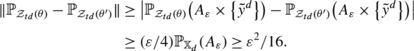Therefore,

$$\mathbb{E} \bigl[ \bigl \vert \mathbb{P}_{\mathbb{Y}_{d}(\theta) | \mathbb {X}_{d}}\bigl( \tilde{y}^{d} | \mathbb{X}_{d}\bigr) - \mathbb{P}_{\mathbb {Y}_{d}(\theta^{\prime})|\mathbb{X}_{d}} \bigl(\tilde{y}^{d} | \mathbb {X}_{d}\bigr)\bigr \vert \bigr] \leq4 \sqrt{ \| \mathbb{P}_{\mathcal{Z}_{t d}(\theta)} - \mathbb {P}_{\mathcal {Z}_{t d}(\theta^{\prime})}\|},$$

which means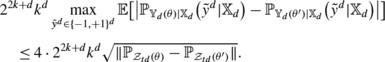□

The following lemma is a standard result on the existence of converging density estimators for totally bounded families of distributions. For our purposes, the details of the estimator achieving this guarantee are not particularly important, as we will apply the result as stated. For completeness, we describe a particular estimator that does achieve the guarantee after the lemma.

### Lemma 4

(Yatracos 1985; Devroye and Lugosi 2001)

Let $$\mathcal{P} = \{ p_{\theta} : \theta\in\varTheta\}$$ be a totally bounded family of probability measures on a measurable space $$(\varOmega,\mathcal{F})$$, and let {W t (θ)} t∈ℕ,θΘ be Ω-valued random variables such that {W t (θ)} t∈ℕ are i.i.d. p θ for each θΘ. Then there exists an estimator $$\hat{\theta}_{T {\theta_{\star}}} = \hat{\theta}_{T}(W_{1}({\theta_{\star}}), \ldots, W_{T}({\theta_{\star}}))$$ and functions $$R_{\mathcal{P}} : \mathbb{N}_{0} \times(0,1] \to [0,\infty)$$ and $$\delta_{\mathcal{P}} : \mathbb{N}_{0} \times(0,1] \to[0,1]$$ such thatα>0, $$\lim_{T \to\infty} R_{\mathcal{P}}(T,\alpha ) = \lim_{T \to\infty} \delta_{\mathcal{P}}(T,\alpha) = 0$$, andθ Θ and T∈ℕ0,

$$\mathbb{P} \bigl( \| p_{\hat{\theta}_{T {\theta_{\star}}}} - p_{{\theta _{\star}}} \| > R_{\mathcal{P}}(T,\alpha) \bigr) \leq\delta_{\mathcal{P}}(T,\alpha) \leq \alpha.$$

In many contexts (though certainly not all), even a simple maximum likelihood estimator suffices to supply this guarantee. However, to derive results under the more general conditions we consider here, we require a more involved method: specifically, the minimum distance skeleton estimate explored by Yatracos (1985), Devroye and Lugosi (2001), specified as follows. Let Θ ε Θ be a minimal-cardinality ε-cover of Θ: that is, a minimal-cardinality subset of Θ such that ∀θΘ, ∃θ ε Θ ε with $$\|p_{\theta_{\varepsilon}} - p_{\theta}\| < \varepsilon$$. For each θ,θ′∈Θ ε , let A θ,θ be a set in $$\mathcal{F}$$ maximizing p θ (A θ,θ)−p θ(A θ,θ), and let $$\mathcal{A}_{\varepsilon} = \{A_{\theta,\theta^{\prime}} : \theta ,\theta^{\prime} \in\varTheta_{\varepsilon}\}$$, known as a Yatracos class. Finally, for $$A \in\mathcal{F}$$, let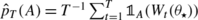. The minimum distance skeleton estimate is $$\hat{\theta}_{T {\theta_{\star}}} = \mathop {\mathrm {argmin}}_{\theta\in\varTheta _{\varepsilon}} \sup_{A \in\mathcal{A}_{\varepsilon}} \vert p_{\theta}(A) - \hat{p}_{T}(A)\vert$$. The reader is referred to Yatracos (1985), Devroye and Lugosi (2001) for a proof that this method satisfies the guarantee of Lemma 4. In particular, if ε T is a sequence decreasing to 0 at a rate such that $$T^{-1} \log(|\varTheta_{\varepsilon_{T}}|) \to0$$, and δ T is a sequence bounded by α and decreasing to 0 with $$\delta_{T} = \omega(\varepsilon_{T} + \sqrt{T^{-1} \log (|\varTheta_{\varepsilon_{T}}|)})$$, then the result of Yatracos (1985), Devroye and Lugosi (2001), combined with Markov’s inequality, implies that to satisfy the condition of Lemma 4, it suffices to take $$R_{\mathcal{P}}(T,\alpha) = \delta_{T}^{-1} ( 3 \varepsilon_{T} + \sqrt{8 T^{-1} \log(2 |\varTheta_{\varepsilon_{T}}|^{2} \lor 8)} )$$ and $$\delta_{\mathcal{P}}(T,\alpha) = \delta_{T}$$. For instance, $$\varepsilon_{T} = 2\inf \{ \varepsilon> 0 : \log (|\varTheta_{\varepsilon}|) \leq\sqrt{T} \}$$ and $$\delta_{T} = \alpha\land(\sqrt{ \varepsilon_{T} } + T^{-1/8})$$ suffice.

We are now ready for the proof of Theorem 1.

### Proof of Theorem 1

For ε>0, let Θ ε Θ be a finite subset such that ∀θΘ, ∃θ ε Θ ε with $$\| \pi_{\theta_{\varepsilon}} - \pi_{\theta}\| < \varepsilon$$; this exists by the assumption that {π θ :θΘ} is totally bounded. Then Lemma 2 implies that ∀θΘ, ∃θ ε Θ ε with $$\|\mathbb{P}_{\mathcal{Z}_{t d}(\theta_{\varepsilon})} - \mathbb{P}_{\mathcal{Z}_{t d}(\theta)} \| \leq\|\pi_{\theta_{\varepsilon}} - \pi_{\theta}\| < \varepsilon$$, so that $$\{\mathbb{P}_{\mathcal{Z}_{t d}(\theta_{\varepsilon})} : \theta_{\varepsilon} \in\varTheta_{\varepsilon}\}$$ is a finite ε-cover of $$\{\mathbb{P}_{\mathcal{Z}_{td}(\theta)} : \theta \in\varTheta\}$$. Therefore, $$\{\mathbb{P}_{\mathcal{Z}_{t d}(\theta)} : \theta\in \varTheta \}$$ is totally bounded. Lemma 4 then implies that there exists an estimator $$\hat{\theta}_{T {\theta_{\star}}} = \hat{\theta}_{T}(\mathcal {Z}_{1 d}({\theta_{\star}}), \ldots, \mathcal{Z}_{T d}({\theta_{\star}}))$$ and functions R d :ℕ0×(0,1]→[0,∞) and δ d :ℕ0×(0,1]→[0,1] such that ∀α>0, lim T→∞ R d (T,α)=lim T→∞ δ d (T,α)=0, and ∀θ Θ and T∈ℕ0,

$$\mathbb{P} \bigl( \| \mathbb{P}_{\mathcal{Z}_{(T+1) d}(\hat{\theta }_{T {\theta _{\star}}}) | \hat{\theta}_{T {\theta_{\star}}}} - \mathbb {P}_{\mathcal {Z}_{(T+1) d}({\theta_{\star}})} \| > R_{d}(T,\alpha) \bigr) \leq \delta_{d}(T,\alpha) \leq\alpha.$$
(2)

Defining

$$R(T,\alpha) = \min_{k \in\mathbb{N}} \bigl(r_{k} + 4 \cdot2^{2k+d} k^{d} \sqrt{R_{d}(T,\alpha)} \bigr),$$

and δ(T,α)=δ d (T,α), and combining (2) with Lemmas 3 and 2, we have

$$\mathbb{P} \bigl( \| \pi_{\hat{\theta}_{T {\theta_{\star}}}} - \pi_{{\theta_{\star}}}\| > R(T,\alpha) \bigr) \leq\delta(T,\alpha) \leq\alpha.$$

Finally, note that lim k→∞ r k =0 and lim T→∞ R d (T,α)=0 imply that lim T→∞ R(T,α)=0. □

### Identifiability from d points

Inspection of the above proof reveals that the assumption that the family of priors is totally bounded is required only to establish the estimability and bounded minimax rate guarantees. In particular, the implied identifiability condition is, in fact, always satisfied, as stated formally in the following corollary.

### Corollary 1

For any priors π 1, π 2 on ℂ, if $$h^{*}_{i} \sim\pi_{i}$$, X 1,…,X d are i.i.d. $$\mathcal{D}$$ independent from $$h^{*}_{i}$$, and $$Z_{d}(i) = \{(X_{1},h^{*}_{i}(X_{1})),\ldots, (X_{d},h^{*}_{i}(X_{d}))\}$$ for i∈{1,2}, then $$\mathbb{P}_{Z_{d}(1)} = \mathbb{P}_{Z_{d}(2)} \implies\pi_{1} = \pi_{2}$$.

### Proof

The described scenario is a special case of our general setting, with Θ={1,2}, in which case $$\mathbb{P}_{Z_{d}(i)} = \mathbb{P}_{\mathcal{Z}_{1 d}(i)}$$. Thus, if $$\mathbb{P}_{Z_{d}(1)} = \mathbb{P}_{Z_{d}(2)}$$, then Lemmas 3 and 2 combine to imply that ∥π 1π 2∥≤inf k∈ℕ r k =0. □

Since Corollary 1 is interesting in itself, it is worth noting that there is a simple direct proof of this result. Specifically, by an inductive argument based on the observation (1) from the proof of Lemma 3, we quickly find that for any k∈ℕ, $$\mathbb {P}_{\mathcal{Z}_{t k}({\theta_{\star}})}$$ is identifiable from $$\mathbb{P}_{\mathcal{Z}_{t d}({\theta_{\star}})}$$. Then we merely recall that $$\mathbb{P}_{\mathcal{Z}_{t}({\theta_{\star}})}$$ is always identifiable from $$\{\mathbb{P}_{\mathcal{Z}_{t k}({\theta_{\star}})} : k \in \mathbb {N}\}$$ (Kallenberg 2002), and the argument from the proof of Lemma 1 shows $$\pi_{{\theta_{\star}}}$$ is identifiable from $$\mathbb{P}_{\mathcal{Z}_{t}({\theta_{\star}})}$$.

It is natural to wonder whether identifiability of $$\pi_{{\theta _{\star}}}$$ from $$\mathbb{P}_{\mathcal{Z}_{t k}({\theta_{\star}})}$$ remains true for some smaller number of points k<d, so that we might hope to create an estimator for $$\pi_{{\theta_{\star}}}$$ based on an estimator for $$\mathbb {P}_{\mathcal {Z}_{t k}({\theta_{\star}})}$$. However, one can show that d is actually the minimum possible value for which this remains true for all $$\mathcal{D}$$ and all families of priors. Formally, we have the following result, holding for every VC class ℂ.

### Theorem 2

There exists a data distribution $$\mathcal{D}$$ and priors π 1,π 2 onsuch that, for any positive integer k<d, if $$h^{*}_{i} \sim\pi_{i}$$, X 1,…,X k are i.i.d. $$\mathcal{D}$$ independent from $$h^{*}_{i}$$, and $$Z_{k}(i) = \{(X_{1},h^{*}_{i}(X_{1})),\ldots ,(X_{k},h^{*}_{i}(X_{k}))\}$$ for i∈{1,2}, then $$\mathbb{P}_{Z_{k}(1)} = \mathbb{P}_{Z_{k}(2)}$$ but π 1π 2.

### Proof

Note that it suffices to show this is the case for k=d−1, since any smaller k is a marginal of this case. Consider a shatterable set of points $$S_{d} = \{x_{1},x_{2},\ldots,x_{d}\} \subseteq\mathcal{X}$$, and let $$\mathcal{D}$$ be uniform on S d . Let ℂ[S d ] be any 2d classifiers in ℂ that shatter S d . Let π 1 be the uniform distribution on ℂ[S]. Now let S d−1={x 1,…,x d−1} and ℂ[S d−1]⊆ℂ[S d ] shatter S d−1 with the property that ∀h∈ℂ[S d−1], $$h(x_{d}) = \prod_{j=1}^{d-1} h(x_{j})$$. Let π 2 be uniform on ℂ[S d−1]. Now for any k<d and distinct indices t 1,…,t k ∈{1,…,d}, $$\{h^{*}_{i}(x_{t_{1}}),\ldots,h^{*}_{i}(x_{t_{k}})\}$$ is distributed uniformly in {−1,+1}k for both i∈{1,2}. This implies $$\mathbb{P}_{Z_{d-1}(1) | X_{1},\ldots,X_{d-1}} = \mathbb {P}_{Z_{d-1}(2) | X_{1},\ldots,X_{d-1}}$$, which implies $$\mathbb{P}_{Z_{d-1}(1)} = \mathbb{P}_{Z_{d-1}(2)}$$. However, π 1 is clearly different from π 2, since even the sizes of the supports are different. □

## Transfer learning

In this section, we look at an application of the techniques from the previous section to transfer learning. Like the previous section, the results in this section are general, in that they are applicable to a variety of learning protocols, including passive supervised learning, passive semi-supervised learning, active learning, and learning with certain general types of data-dependent interaction (see Hanneke 2009). For simplicity, we restrict our discussion to the active learning formulation; the analogous results for these other learning protocols follow by similar reasoning.

The result of the previous section implies that an estimator for θ based on d-dimensional joint distributions is consistent with a bounded rate of convergence R. Therefore, for certain prior-dependent learning algorithms, their behavior should be similar under $$\pi_{\hat{\theta}_{T {\theta_{\star}}}}$$ to their behavior under $$\pi_{{\theta_{\star}}}$$.

To make this concrete, we formalize this in the active learning protocol as follows. A prior-dependent active learning algorithm $$\mathcal{A}$$ takes as inputs ε>0, $$\mathcal{D}$$, and a distribution π on ℂ. It initially has access to X 1,X 2,… i.i.d. $$\mathcal{D}$$; it then selects an index i 1 to request the label for, receives $$Y_{i_{1}} = h^{*}(X_{i_{1}})$$, then selects another index i 2, etc., until it eventually terminates and returns a classifier. Denote by $$\mathcal{Z}= \{(X_{1},h^{*}(X_{1})),(X_{2},h^{*}(X_{2})),\ldots\}$$. To be correct, $$\mathcal{A}$$ must guarantee that for h π, ∀ε>0, $$\mathbb{E} [\rho(\mathcal {A}(\varepsilon, \mathcal{D}, \pi), h^{*}) ] \leq\varepsilon$$. We define the random variable $$N(\mathcal{A},f,\varepsilon,\mathcal {D},\pi)$$ as the number of label requests $$\mathcal{A}$$ makes before terminating, when given ε, $$\mathcal{D}$$, and π as inputs, and when h =f is the value of the target function; we make the particular data sequence $$\mathcal {Z}$$ the algorithm is run with implicit in this notation. We will be interested in the expected sample complexity $$SC(\mathcal{A},\varepsilon,\mathcal{D},\pi) = \mathbb{E} [ N(\mathcal {A},h^{*},\varepsilon,\mathcal{D},\pi) ]$$.

We propose the following algorithm $$\mathcal{A}_{\tau}$$ for transfer learning, defined in terms of a given correct prior-dependent active learning algorithm $$\mathcal{A}_{a}$$. We discuss interesting specifications for $$\mathcal{A}_{a}$$ in the next section, but for now the only assumption we require is that for any ε>0 and $$\mathcal{D}$$, there is a value s ε <∞ such that for every π and f∈ℂ, $$N(\mathcal{A}_{a}, f, \varepsilon, \mathcal{D}, \pi) \leq s_{\varepsilon }$$; this is a very mild requirement, and any active learning algorithm can be converted into one that satisfies this without significantly increasing its sample complexities for the priors it is already good for Balcan et al. (2010). We additionally denote by $$m_{\varepsilon} = \frac{16 d}{\varepsilon} \ln (\frac {24}{\varepsilon} )$$, and B(θ,γ)={θ′∈Θ:∥π θ π θ∥≤γ}.

Recall that $$\hat{\theta}_{(t-1) {\theta_{\star}}}$$, which is defined by Theorem 1, is a function of the labels requested on previous rounds of the algorithm; R(t−1,ε/2) is also defined by Theorem 1, and has no dependence on the data (or on θ ). The other quantities referred to in Algorithm 1 are defined just prior to Algorithm 1. We suppose the algorithm has access to the value $$SC(\mathcal{A}_{a}, \varepsilon/4, \mathcal{D}, \pi_{\theta})$$ for every θΘ. This can sometimes be calculated analytically as a function of θ, or else can typically be approximated via Monte Carlo simulations. In fact, the result below holds even if SC is merely an accessible upper bound on the expected sample complexity.Algorithm 1

### Theorem 3

The algorithm $$\mathcal{A}_{\tau}$$ is correct. Furthermore, if S T (ε) is the total number of label requests made by $$\mathcal{A}_{\tau}(T,\varepsilon)$$, then $$\limsup_{T \to\infty} \frac{\mathbb{E}[S_{T}(\varepsilon)]}{T} \leq SC(\mathcal{A}_{a}, \varepsilon/4, \mathcal{D}, \pi_{{\theta_{\star}}}) + d$$.

The implication of Theorem 3 is that, via transfer learning, it is possible to achieve almost the same long-run average sample complexity as would be achievable if the target’s prior distribution were known to the learner. We will see in the next section that this is sometimes significantly better than the single-task sample complexity. As mentioned, results of this type for transfer learning have previously appeared when $$\mathcal{A}_{a}$$ is a passive learning method (Baxter 1997); however, to our knowledge, this is the first such result where the asymptotics concern only the number of learning tasks, not the number of samples per task; this is also the first result we know of that is immediately applicable to more sophisticated learning protocols such as active learning.

The algorithm $$\mathcal{A}_{\tau}$$ is stated in a simple way here, but Theorem 3 can be improved with some obvious modifications to $$\mathcal{A}_{\tau}$$. The extra “+d” in Theorem 3 is not actually necessary, since we could stop updating the estimator $$\check{\theta}_{t {\theta _{\star}}}$$ (and the corresponding R value) after some o(T) number of rounds (e.g., $$\sqrt{T}$$), in which case we would not need to request Y t1(θ ),…,Y td (θ ) for t larger than this, and the extra do(T) number of labeled examples vanishes in the average as T→∞. Additionally, the ε/4 term can easily be improved to any value arbitrarily close to ε (even (1−o(1))ε) by running $$\mathcal{A}_{a}$$ with argument ε−2R(t−1,ε/2)−δ(t−1,ε/2) instead of ε/4, and using this value in the SC calculations in the definition of $$\check{\theta}_{t {\theta_{\star}}}$$ as well. In fact, for many algorithms $$\mathcal{A}_{a}$$ (e.g., with $$SC(\mathcal{A}_{a},\varepsilon,\mathcal{D},\pi_{{\theta_{\star}}})$$ continuous in ε), combining the above two tricks yields $$\limsup_{T \to\infty} \frac{\mathbb{E}[S_{T}(\varepsilon )]}{T} \leq SC(\mathcal{A}_{a}, \varepsilon, \mathcal{D}, \pi_{{\theta _{\star}}})$$.

Returning to our motivational remarks from Sect. 2.1, we can ask how many extra labeled examples are required from each learning problem to gain the benefits of transfer learning. This question essentially concerns the initial step of requesting the labels Y t1(θ ),…,Y td (θ ). Clearly this indicates that from each learning problem, we need at most d extra labeled examples to gain the benefits of transfer. Whether these d label requests are indeed extra depends on the particular learning algorithm $$\mathcal{A}_{a}$$; that is, in some cases (e.g., certain passive learning algorithms), $$\mathcal {A}_{a}$$ may itself use these initial d labels for learning, so that in these cases the benefits of transfer learning are essentially gained as a by-product of the learning processes, and essentially no additional labeling effort need be expended to gain these benefits. On the other hand, for some active learning algorithms, we may expect that at least some of these initial d labels would not be requested by the algorithm, so that some extra labeling effort is expended to gain the benefits of transfer in these cases.

One drawback of our approach is that we require the data distribution $$\mathcal{D}$$ to remain fixed across tasks (this contrasts with Baxter 1997). However, it should be possible to relax this requirement in the active learning setting in many cases. For instance, if $$\mathcal{X}= \mathbb {R}^{k}$$, then as long as we are guaranteed that the distribution $$\mathcal{D}_{t}$$ for each learning task has a strictly positive density function, it should be possible to use rejection sampling for each task to guarantee the d queried examples from each task have approximately the same distribution across tasks. This is all we require for our consistency results on $$\hat{\theta}_{T {\theta_{\star}}}$$ (i.e., it was not important that the d samples came from the true distribution $$\mathcal{D}$$, only that they came from a distribution under which ρ is a metric). We leave the details of such an adaptive method for future consideration.

### Proof of Theorem 3

Recall that, to establish correctness, we must show that ∀tT, $$\mathbb{E} [ \rho (\hat{h}_{t}, h^{*}_{t {\theta_{\star}}} ) ] \leq\varepsilon$$, regardless of the value of θ Θ. Fix any θ Θ and tT. If R(t−1,ε/2)>ε/8, then classic results from passive learning indicate that $$\mathbb{E} [ \rho (\hat {h}_{t}, h^{*}_{t {\theta_{\star}}} ) ] \leq\varepsilon$$ (Vapnik 1982). Otherwise, by Theorem 1, with probability at least 1−ε/2, we have $$\| \pi_{{\theta_{\star}}} - \pi_{\hat {\theta}_{(t-1) {\theta_{\star}}}}\| \leq R(t-1,\varepsilon/2)$$. On this event, if R(t−1,ε/2)≤ε/8, then by a triangle inequality $$\|\pi_{\check{\theta}_{t {\theta_{\star}}}} - \pi_{{\theta _{\star}}}\| \leq2 R(t-1,\varepsilon/2) \leq\varepsilon/ 4$$. Thus,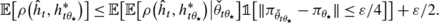(3)

For θΘ, let $$\hat{h}_{t \theta}$$ denote the classifier that would be returned by $$\mathcal{A}_{a}(\varepsilon/4, \mathcal{D}, \pi_{\check{\theta}_{t {\theta_{\star}}}})$$ when run with data sequence $$\{(X_{t 1}, h^{*}_{t \theta}(X_{t 1})), (X_{t 2}, h^{*}_{t \theta}(X_{t 2})), \ldots\}$$. Note that for any θΘ, any measurable function F:ℂ→[0,1] has

$$\mathbb{E} \bigl[ F\bigl(h^{*}_{t {\theta_{\star}}} \bigr) \bigr] \leq\mathbb {E} \bigl[ F\bigl(h^{*}_{t \theta}\bigr) \bigr] + \| \pi_{\theta} - \pi_{{\theta_{\star}}}\|.$$
(4)

In particular, supposing $$\| \pi_{\check{\theta}_{t {\theta_{\star }}}} - \pi_{{\theta_{\star}}}\| \leq\varepsilon/4$$, we have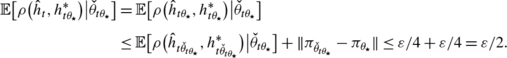Combined with (3), this implies $$\mathbb {E} [\rho (\hat{h}_{t},h^{*}_{t {\theta_{\star}}} ) ] \leq\varepsilon$$.

We establish the sample complexity claim as follows. First note that convergence of R(t−1,ε/2) implies that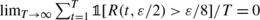, and that the number of labels used for a value of t with R(t−1,ε/2)>ε/8 is bounded by a finite function m ε of ε. Therefore,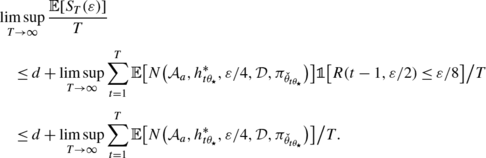(5)

By the definition of R,δ from Theorem 1, we have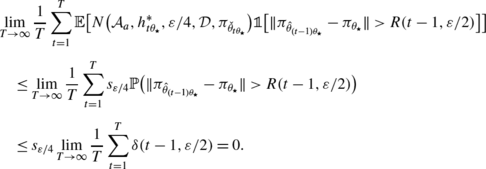Combined with (5), this implies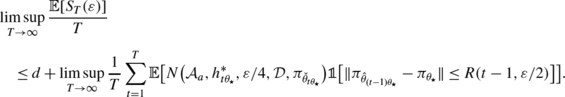For any tT, on the event $$\| \pi_{\hat{\theta}_{(t-1) {\theta_{\star}}}} - \pi_{{\theta_{\star}}}\| \leq R(t-1, \varepsilon/2)$$, we have (by the property (4) and a triangle inequality)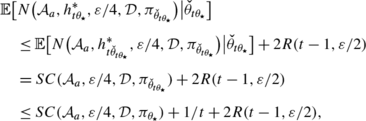where the last inequality follows by definition of $$\check{\theta}_{t {\theta_{\star}}}$$. Therefore,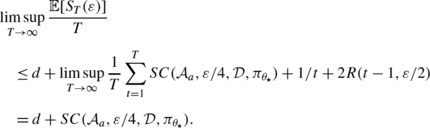□

## Application to self-verifying active learning

In this section, we examine a specific sample complexity guarantee achievable by active learning, when combined with the above transfer learning procedure. As mentioned, Balcan et al. (2010) found that there is often a significant gap between the sample complexities achievable by good active learning algorithms in general and the sample complexities achievable by active learning algorithms that themselves adaptively determine how many label requests to make for any given problem (referred to as self-verifying active learning algorithms). Specifically, while the former can always be strictly superior to the sample complexities achievable by passive learning, there are simple examples where this is not the case for self-verifying active learning algorithms.

We should note, however, that all of the above considerations were proven for a learning scenario in which the target concept is considered a constant, and no information about the process that generates this concept is available to the learner. Thus, there is a natural question of whether, in the context of the transfer learning setting described above, we might be able to close this gap, so that self-verifying active learning algorithms are able to achieve the same type of guaranteed strict improvements over passive learning that are achievable by their non-self-verifying counterparts.

The considerations of the previous section indicate that this question is in some sense reducible to an analogous question for prior-dependent self-verifying active learning algorithms. The quantity $$SC(\mathcal{A}_{a}, \varepsilon,/4, \mathcal{D}, \pi_{{\theta_{\star}}})$$ then essentially characterizes the achievable average sample complexity among the sequence of tasks. We will therefore focus in this section on characterizing this quantity, for a particularly effective active learning algorithm $$\mathcal{A}_{a}$$.

Throughout this section, we suppose the active learning algorithm $$\mathcal{A}_{a}$$ is run with the sequence of examples $$\mathcal{Z}= \{(X_{1}, h^{*}(X_{1})), (X_{2}, h^{*}(X_{2})), \ldots\}$$, where X 1,X 2,… are i.i.d. $$\mathcal{D}$$, and $$h^{*}\sim\pi_{{\theta _{\star}}}$$.

### Related work on prior-dependent learning

Prior-dependent learning algorithms have been studied in depth in the context of passive learning. In particular, Haussler et al. (1992) found that for any concept space of finite VC dimension d, for any prior and data distribution, O(d/ε) random labeled examples are sufficient for the expected error rate of the Bayes classifier produced under the posterior distribution to be at most ε. Furthermore, it is easy to construct learning problems for which there is an Ω(1/ε) lower bound on the number of random labeled examples required to achieve expected error rate at most ε, by any passive learning algorithm; for instance, the problem of learning threshold classifiers on [0,1] under a uniform data distribution and uniform prior is one such scenario.

In contrast, a relatively small amount is known about prior-dependent active learning. Freund et al. (1997) analyze the Query By Committee algorithm in this context, and find that if a certain information gain quantity for the points requested by the algorithm is lower-bounded by a value g, then the algorithm requires only O((d/g)log(1/ε)) labels to achieve expected error rate at most ε. In particular, they show that this is satisfied for constant g for linear separators under a near-uniform prior, and a near-uniform data distribution over the unit sphere. This represents a marked improvement over the results of Haussler et al. (1992) for passive learning, and since the Query by Committee algorithm is self-verifying, this result is highly relevant to the present discussion. However, the condition that the information gains be lower-bounded by a constant is quite restrictive, and many interesting learning problems are precluded by this requirement. Furthermore, there exist learning problems (with finite VC dimension) for which the Query by Committee algorithm makes an expected number of label requests exceeding Ω(1/ε). To date, there has not been a general analysis of how the value of g can behave as a function of ε, though such an analysis would likely be quite interesting.

In the present section, we take a more general approach to the question of prior-dependent active learning. We are interested in the broad question of whether access to the prior bridges the gap between the sample complexity of learning and the sample complexity of learning with verification. Specifically, we ask the following question.

Can a prior-dependent self-terminating active learning algorithm for a concept class of finite VC dimension always achieve expected error rate at most ε using o(1/ε) label requests?

### Prior-independent learning algorithms

One may initially wonder whether we could achieve this o(1/ε) result merely by calculating the expected sample complexity of some prior-independent method, thus precluding the need for novel algorithms. Formally, we say an algorithm $$\mathcal{A}$$ is prior-independent if the conditional distribution of the queries and return value of $$\mathcal{A}(\varepsilon,\mathcal {D},\pi )$$ given $$\mathcal{Z}$$ is functionally independent of π. Indeed, for some ℂ and $$\mathcal{D}$$, it is known that there are prior-independent active learning algorithms $$\mathcal{A}$$ that have $$\mathbb{E}[N(\mathcal{A},h^{*},\varepsilon ,\mathcal{D},\pi)|h^{*}] = o(1/\varepsilon)$$ (always); for instance, threshold classifiers have this property under any $$\mathcal{D}$$, homogeneous linear separators have this property under a uniform $$\mathcal{D}$$ on the unit sphere in k dimensions, and intervals with positive width on $$\mathcal{X}=[0,1]$$ have this property under $$\mathcal{D}= \mathrm{Uniform}([0,1])$$ (see e.g., Dasgupta 2005). It is straightforward to show that any such $$\mathcal{A}$$ will also have $$SC(\mathcal{A},\varepsilon ,\mathcal{D} ,\pi) = o(1/\varepsilon)$$ for every π. In particular, the law of total expectation and the dominated convergence theorem imply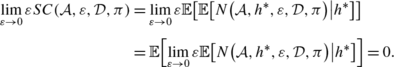In these cases, we can think of SC as a kind of average-case analysis of these algorithms. However, as we discuss next, there are also many ℂ and $$\mathcal{D}$$ for which there is no prior-independent algorithm achieving o(1/ε) sample complexity for all priors. Thus, any general result on o(1/ε) expected sample complexity for π-dependent algorithms would indicate that there is a real advantage to having access to the prior, beyond the apparent smoothing effects of an average-case analysis.

As an example of a problem where no prior-independent self-verifying algorithm can achieve o(1/ε) sample complexity, consider $$\mathcal{X}= [0,1]$$, $$\mathcal{D}= \mathrm{Uniform}([0,1])$$, and ℂ as the concept space of interval classifiers: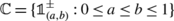, whereif x∈(a,b) and −1 otherwise. Note that because we allow a=b, there is a classifier h ∈ℂ labeling all of $$\mathcal{X}$$ negative. For 0≤ab≤1, let π (a,b) denote the prior with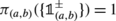. We now show any correct prior-independent algorithm has Ω(1/ε) sample complexity for π (0,0), following a technique of Balcan et al. (2010). Consider any ε∈(0,1/144) and any prior-independent active learning algorithm $$\mathcal{A}$$ with $$SC(\mathcal{A},\varepsilon,\mathcal{D},\pi_{(0,0)}) < s = \frac {1}{144\varepsilon}$$. Then define $$H_{\varepsilon} = \{ ( 12 i \varepsilon, 12(i+1)\varepsilon) : i \in \{0,1,\ldots, \lfloor\frac {1-12\varepsilon}{12\varepsilon} \rfloor \} \}$$. Let $$\hat{h}_{(a,b)}$$ denote the classifier returned by $$\mathcal {A}(\varepsilon,\mathcal{D},\cdot)$$ when queries are answered with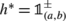, for 0≤ab≤1, and let R (a,b) denote the set of examples (x,y) for which $$\mathcal{A}(\varepsilon,\mathcal{D},\cdot)$$ requests labels (including their y=h (x) labels). The point of this construction is that, with such a small number of queries, for many of the (a,b)∈H ε , the algorithm must behave identically foras for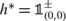(i.e., R (a,b)=R (0,0), and hence $$\hat{h}_{(a,b)} = \hat{h}_{(0,0)}$$). These π (a,b) priors will then witness the fact that $$\mathcal {A}$$ is not a correct self-verifying algorithm. Formally,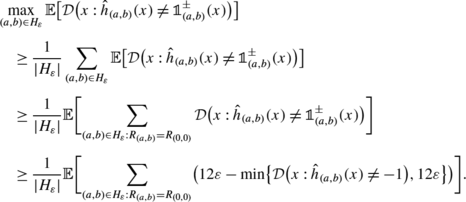(6)

Since the summation in (6) is restricted to (a,b) with R (a,b)=R (0,0), these (a,b) must also have $$\hat{h}_{(a,b)} = \hat{h}_{(0,0)}$$, so that (6) equals

$$\frac{1}{|H_{\varepsilon}|} \mathbb{E} \biggl[ \sum _{(a,b) \in H_{\varepsilon} : R_{(a,b)}=R_{(0,0)}} \bigl(12 \varepsilon- \min\bigl\{ \mathcal{D} \bigl(x : \hat{h}_{(0,0)}(x) \neq-1\bigr), 12\varepsilon\bigr\} \bigr) \biggr].$$
(7)

Furthermore, for a given $$\mathcal{Z}$$ sequence, the only (a,b)∈H ε with R (a,b)R (0,0) are those for which some (x,−1)∈R (0,0) has x∈(a,b); since the (a,b)∈H ε are disjoint, the above summation has at least |H ε |−|R (0,0)| elements in it. Thus, (7) is at least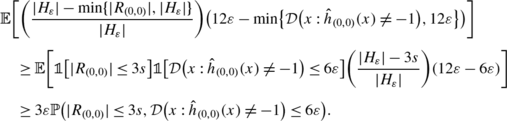(8)

By Markov’s inequality,

$$\mathbb{P} \bigl(|R_{(0,0)}| > 3s \bigr) \leq\mathbb {E}\bigl[|R_{(0,0)}|\bigr] / (3s) = SC(\mathcal{A},\varepsilon,\mathcal{D},\pi_{(0,0)}) / (3s) < 1/3,$$

and $$\mathbb{P} ( \mathcal{D}(x : \hat{h}_{(0,0)}(x) \neq-1) > 6 \varepsilon ) \leq\mathbb{E} [ \mathcal{D}(x : \hat{h}_{(0,0)}(x) \neq-1) ] / (6 \varepsilon)$$, and if $$\mathcal{A}$$ is a correct self-verifying algorithm, then $$\mathbb{E} [ \mathcal{D}(x : \hat{h}_{(0,0)}(x) \neq-1) ] / (6 \varepsilon) \leq1/6$$. Thus, by a union bound, (8) is at least 3ε(1−1/3−1/6)=(3/2)ε>ε. Therefore, $$\mathcal{A}$$ cannot be a correct self-verifying learning algorithm.

### Prior-dependent learning: an example

We begin our exploration of π-dependent active learning with a concrete example, namely interval classifiers under a uniform data density but arbitrary prior, to illustrate how access to the prior can make a difference in the sample complexity. Specifically, consider $$\mathcal{X}= [0,1]$$, $$\mathcal{D}$$ uniform on [0,1], and the concept space ℂ of interval classifiers specified in the previous subsection. For each classifier h∈ℂ, define $$w(h) = \mathcal{D}(x : h(x) = +1)$$ (the width of the interval h). Note that because we allow a=b in the definition of ℂ, there is a classifier h ∈ℂ with w(h )=0.

For simplicity, in this example (only) we will suppose the algorithm may request the label of any point in $$\mathcal{X}$$, not just those in the sequence {X i }; the same ideas can easily be adapted to the setting where queries are restricted to {X i }. Consider an active learning algorithm that sequentially requests the labels h (x) for points x at 1/2, 1/4, 3/4, 1/8, 3/8, 5/8, 7/8, 1/16, 3/16, etc., until (case 1) it encounters an example x with h (x)=+1 or until (case 2) the set of classifiers V⊆ℂ consistent with all observed labels so far satisfies $$\mathbb{E}[ w(h^{*}) | V] \leq\varepsilon$$ (which ever comes first). In case 2, the algorithm simply halts and returns the constant classifier that always predicts −1: call it h ; note that ρ(h ,h )=w(h ). In case 1, the algorithm enters a second phase, in which it performs a binary search (repeatedly querying the midpoint between the closest two −1 and +1 points, taking 0 and 1 as known negative points) to the left and right of the observed positive point, halting after log2(4/ε) label requests on each side; this results in estimates of the target’s endpoints up to ±ε/4, so that returning any classifier among the set V⊆ℂ consistent with these labels results in error rate at most ε; in particular, if $$\tilde{h}$$ is the classifier in V returned, then $$\mathbb{E}[ \rho(\tilde{h},h^{*}) | V] \leq\varepsilon$$.

Denoting this algorithm by $$\mathcal{A}_{[]}$$, and $$\hat{h}$$ the classifier it returns, we have

$$\mathbb{E} \bigl[ \rho \bigl( \hat{h}, h^{*} \bigr) \bigr] = \mathbb{E} \bigl[ \mathbb{E} \bigl[ \rho \bigl(\hat {h},h^{*} \bigr) \big| V \bigr] \bigr] \leq\varepsilon,$$

so that the algorithm is definitely correct.

Note that case 2 will definitely be satisfied after at most $$\frac {2}{\varepsilon}$$ label requests, and if w(h )>ε, then case 1 will definitely be satisfied after at most $$\frac{2}{w(h^{*})}$$ label requests, so that the algorithm never makes more than $$\frac{2}{\max\{ w(h^{*}),\varepsilon\}}$$ label requests before satisfying one of the two cases. Abbreviating $$N(h^{*}) = N(\mathcal{A}_{[]}, h^{*},\varepsilon ,\mathcal{D} ,\pi)$$, we have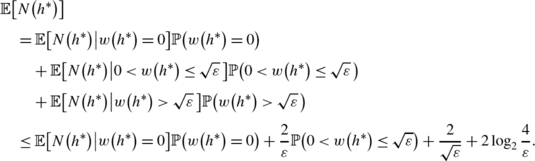(9)

The third and fourth terms in (9) are o(1/ε). Since $$\mathbb{P}(0<w(h^{*})\leq\sqrt{\varepsilon}) \to0$$ as ε→0, the second term in (9) is o(1/ε) as well. If ℙ(w(h )=0)=0, this completes the proof. We focus the rest of the proof on the first term in (9), in the case that ℙ(w(h )=0)>0: i.e., there is nonzero probability that the target h labels the space all negative. Letting V denote the subset of ℂ consistent with all requested labels, note that on the event w(h )=0, after n label requests (for n+1 a power of 2) we have max hV w(h)≤1/n. Thus, for any value γ∈(0,1), after at most $$\frac{2}{\gamma }$$ label requests, on the event that w(h )=0,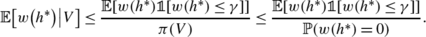(10)

Now note that, by the dominated convergence theorem,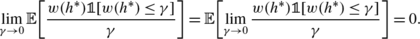Therefore,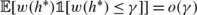. If we define γ ε as the largest value of γ for which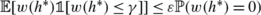(or, say, half the supremum if the maximum is not achieved), then we have γ ε ε. Combined with (10), this implies

$$\mathbb{E} \bigl[N\bigl(h^{*}\bigr) \big| w\bigl(h^{*}\bigr) = 0 \bigr] \leq\frac {2}{\gamma_{\varepsilon}} = o(1/\varepsilon).$$

Thus, all of the terms in (9) are o(1/ε), so that in total $$\mathbb{E}[N(h^{*})] = o(1/\varepsilon)$$.

In conclusion, for this concept space ℂ and data distribution $$\mathcal{D}$$, we have a correct active learning algorithm $$\mathcal{A}$$ achieving a sample complexity $$SC(\mathcal{A}, \varepsilon, \mathcal{D}, \pi) = o(1/\varepsilon )$$ for all priors π on ℂ.

### A general result for self-verifying Bayesian active learning

In this subsection, we present our main result for improvements achievable by prior-dependent self-verifying active learning: a general result stating that o(1/ε) expected sample complexity is always achievable for some appropriate prior-dependent active learning algorithm, for any $$(\mathcal{X},\mathbb{C},\mathcal{D},\pi)$$ for which ℂ has finite VC dimension. Since the known results for the sample complexity of passive learning with access to the prior are typically Θ(1/ε) (Haussler et al. 1992), and since there are known learning problems $$(\mathcal{X},\mathbb{C},\mathcal{D},\pi)$$ for which every passive learning algorithm requires Ω(1/ε) samples, this o(1/ε) result for active learning represents an improvement over passive learning.

The proof is simple and accessible, yet represents an important step in understanding the problem of self-termination in active learning algorithms, and the general issue of the complexity of verification. Also, since there are problems $$(\mathcal{X},\mathbb{C},\mathcal {D})$$ where ℂ has finite VC dimension but for which no (single-task) prior-independent correct active learning algorithm (of the self-terminating type studied here) can achieve o(1/ε) expected sample complexity for every π, this also represents a significant step toward understanding the inherent value of having access to the prior in active learning. Additionally, via Theorem 3, this result implies that active transfer learning (of the type discussed above) can provide strictly superior sample complexities compared to the known results for passive learning (even compared to passive learning algorithms having direct access to the prior $$\pi_{{\theta_{\star}}}$$), and often strictly superior to the sample complexities achievable by (prior-independent) active learning without transfer.

First, we have a small lemma.

### Lemma 5

For any sequence of functions ϕ n :ℂ→[0,∞) such that, ∀f∈ℂ, ϕ n (f)=o(1/n) andn∈ℕ, ϕ n (f)≤c/n (for an f-independent constant c∈(0,∞)), there exists a sequence $$\bar{\phi}_{n}$$ in [0,∞) such that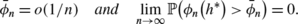### Proof

For any constant γ∈(0,∞), we have (by Markov’s inequality and the dominated convergence theorem)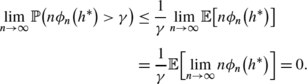Therefore (by induction), there exists a diverging sequence n i in ℕ such that

$$\lim_{i \to\infty} \sup_{n \geq n_i} \mathbb{P} \bigl(n\phi_n \bigl(h^{*}\bigr) > 2^{-i} \bigr) = 0.$$

Inverting this, let i n =max{i∈ℕ:n i n}, and define $$\bar{\phi}_{n}(h^{*}) = (1/n) \cdot2^{-i_{n}}$$. By construction, $$\mathbb{P} (\phi_{n}(h^{*}) > \bar{\phi }_{n} ) \to0$$. Furthermore, $$n_{i} \to\infty\implies i_{n} \to\infty$$, so that we have

$$\lim_{n\to\infty} n \bar{\phi}_n = \lim_{n\to\infty} 2^{-i_n} = 0,$$

implying $$\bar{\phi}_{n} = o(1/n)$$. □

### Theorem 4

For any VC class ℂ, there is a correct active learning algorithm $$\mathcal{A}_{a}$$ that, for every data distribution $$\mathcal{D}$$ and prior π, achieves expected sample complexity

$$SC(\mathcal{A}_{a}, \varepsilon,\mathcal{D},\pi) = o(1/\varepsilon).$$

Our approach to proving Theorem 4 is via a reduction to established results about (prior-independent) active learning algorithms that are not self-verifying. Specifically, consider a slightly different type of active learning algorithm than that defined above: namely, an algorithm $$\mathcal{A}_{b}$$ that takes as input a budget n∈ℕ on the number of label requests it is allowed to make, and that after making at most n label requests returns as output a classifier $$\hat{h}_{n}$$. Let us refer to any such algorithm as a budget-based active learning algorithm. Note that budget-based active learning algorithms are prior-independent (have no direct access to the prior). The following result was proven by Hanneke (2009) (see also the related earlier work of Balcan et al. 2010).

### Lemma 6

(Hanneke 2009)

For any VC class ℂ, there exists a constant c∈(0,∞), a function $$\mathcal{E}(n ; f, \mathcal{D})$$, and a budget-based active learning algorithm $$\mathcal{A}_{b}$$ such that

$$\forall\mathcal{D}, \forall f \in\mathbb{C},\quad \mathcal{E}(n ; f,\mathcal{D}) \leq c/n\quad \text{\textit{and}}\quad \mathcal{E}(n ; f,\mathcal{D}) = o(1/n),$$

and $$\mathbb{E} [ \rho ( \mathcal{A}_{b}(n), h^{*} ) | h^{*} ] \leq\mathcal{E}(n ; h^{*}, \mathcal{D})$$ (always).Footnote 1

That is, equivalently, for any fixed value for the target function, the expected error rate is o(1/n), where the random variable in the expectation is only the data sequence X 1,X 2,… . Our task in the proof of Theorem 4 is to convert such a budget-based algorithm into one that is correct, self-terminating, and prior-dependent, taking ε as input.

### Proof of Theorem 4

Consider $$\mathcal{A}_{b}$$, $$\mathcal{E}$$, and c as in Lemma 6, let $$\hat{h}_{n}$$ denote the classifier returned by $$\mathcal{A}_{b}(n)$$, and define

$$n_{\pi, \varepsilon} = \min \bigl\{ n \in\mathbb{N}: \mathbb {E} \bigl[ \rho \bigl( \hat{h}_{n}, h^{*} \bigr) \bigr] \leq \varepsilon \bigr\}.$$

This value is accessible based purely on access to π and $$\mathcal{D}$$. Furthermore, we clearly have (by construction) $$\mathbb{E} [ \rho (\hat{h}_{n_{\pi,\varepsilon}}, h^{*} ) ] \leq\varepsilon$$. Thus, letting $$\mathcal {A}_{a}$$ denote the active learning algorithm taking $$(\mathcal{D},\pi,\varepsilon)$$ as input, which runs $$\mathcal{A}_{b}(n_{\pi,\varepsilon})$$ and then returns $$\hat{h}_{n_{\pi,\varepsilon}}$$, we have that $$\mathcal{A}_{a}$$ is a correct learning algorithm (i.e., its expected error rate is at most ε).

As for the expected sample complexity $$SC(\mathcal{A}_{a}, \varepsilon ,\mathcal{D},\pi)$$ achieved by $$\mathcal{A}_{a}$$, we have $$SC(\mathcal {A}_{a}, \varepsilon,\mathcal{D},\pi) \leq n_{\pi,\varepsilon}$$, so that it remains only to bound n π,ε . By Lemma 5, there is a π-dependent function $$\mathcal{E}(n ; \pi, \mathcal{D})$$ such that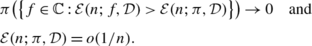Therefore, by the law of total expectation,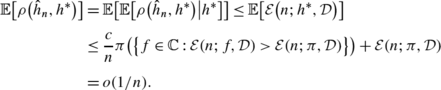If n π,ε =O(1), then clearly n π,ε =o(1/ε) as needed. Otherwise, since n π,ε is monotonic in ε, we must have n π,ε ↑∞ as ε↓0. In particular, in this latter case we have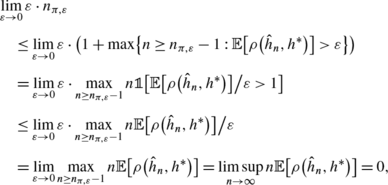so that n π,ε =o(1/ε), as required. □

Theorem 4 implies that, if we have direct access to the prior distribution of h , regardless of what that prior distribution π is, we can always construct a self-verifying active learning algorithm $$\mathcal {A}_{a}$$ that has a guarantee of $$\mathbb{E} [\rho (\mathcal{A}_{a}(\varepsilon ,\mathcal{D},\pi ), h^{*} ) ] \leq\varepsilon$$ and its expected number of label requests is o(1/ε). This guarantee is not possible for prior-independent (single-task) self-verifying active learning algorithms.

Additionally, when combined with Theorem 3, Theorem 4 implies that $$\mathcal{A}_{\tau}$$, with this particular algorithm $$\mathcal{A}_{a}$$ as its subroutine, has $$\limsup_{T \to\infty} \mathbb{E}[S_{T}(\varepsilon)] / T = o(1/\varepsilon)$$. Again, since there are known cases where there is no prior-independent self-verifying active learning algorithm with sample complexity o(1/ε), this sometimes represents a significant improvement over the results provable for learning the tasks independently (i.e., without transfer).

### Dependence on $$\mathcal{D}$$ in the learning algorithm

The dependence on $$\mathcal{D}$$ in the algorithm described in the proof of Theorem 4 is fairly weak, and we can eliminate any direct dependence on $$\mathcal{D}$$ by replacing $$\rho (\hat {h}_{n}, h^{*} )$$ by a 1−ε/2 confidence upper bound based on $$M_{\varepsilon} = \varOmega (\frac{1}{\varepsilon^{2}}\log \frac{1}{\varepsilon} )$$ i.i.d. unlabeled examples $$X_{1}^{\prime }, X_{2}^{\prime}, \ldots, X_{M_{\varepsilon}}^{\prime}$$ independent from the examples used by the algorithm (e.g., set aside in a pre-processing step, where the bound is calculated via Hoeffding’s inequality and a union bound over the values of n that we check, of which there are at most O(1/ε)). Then we simply increase the value of n (starting at some constant, such as 1) until

$$\frac{1}{M_{\varepsilon}} \sum_{i=1}^{M_{\varepsilon}} \pi \bigl( \bigl\{ f \in\mathbb{C}: f \bigl(X_{i}^{\prime} \bigr) \neq \hat {h}_{n} \bigl(X_{i}^{\prime} \bigr) \bigr\} \bigr) \leq\varepsilon / 2.$$

The expected value of the smallest value of n for which this occurs is o(1/ε). Note that this only requires access to the prior π, not the data distribution $$\mathcal{D}$$ (the budget-based algorithm $$\mathcal{A}_{b}$$ of Hanneke (2009) has no direct dependence on $$\mathcal{D}$$); if desired for computational efficiency, this dependence may also be estimated by a 1−ε/4 confidence upper bound based on $$\varOmega (\frac{1}{\varepsilon^{2}}\log\frac {1}{\varepsilon} )$$ independent samples of h values with distribution π, where for each sample we simulate the execution of $$\mathcal{A}_{b}(n)$$ for that (simulated) target function in order to obtain the returned classifier. In particular, note that no actual label requests to the oracle are required during this process of estimating the appropriate label budget n π,ε , as all executions of $$\mathcal{A}_{b}$$ are simulated.

### Inherent dependence on π in the sample complexity

We have shown that for every prior π, the sample complexity is bounded by a o(1/ε) function. One might wonder whether it is possible that the asymptotic dependence on ε in the sample complexity can be prior-independent, while still being o(1/ε). That is, we can ask whether there exists a (π-independent) function s(ε)=o(1/ε) such that, for every π, there is a correct π-dependent algorithm $$\mathcal{A}$$ achieving a sample complexity $$SC(\mathcal{A}, \varepsilon, \mathcal{D}, \pi) = O(s(\varepsilon))$$, possibly involving π-dependent constants. Certainly in some cases, such as threshold classifiers, this is true. However, it seems this is not generally the case, and in particular it fails to hold for the space of interval classifiers.

For instance, consider a prior π on the space ℂ of interval classifiers, constructed as follows. We are given an arbitrary monotonic g(ε)=o(1/ε); since g(ε)=o(1/ε), there must exist (nonzero) functions q 1(i) and q 2(i) such that lim i→∞ q 1(i)=0, lim i→∞ q 2(i)=0, and ∀i∈ℕ,g(q 1(i)/2i+1)≤q 2(i)⋅2i; furthermore, letting q(i)=max{q 1(i),q 2(i)}, by monotonicity of g we also have ∀i∈ℕ,g(q(i)/2i+1)≤q(i)⋅2i, and lim i→∞ q(i)=0. Then define a function p(i) with ∑ i∈ℕ p(i)=1 such that p(i)≥q(i) for infinitely many i∈ℕ; for instance, this can be done inductively as follows. Let α 0=1/2; for each i∈ℕ, if q(i)>α i−1, set p(i)=0 and α i =α i−1; otherwise, set p(i)=α i−1 and α i =α i−1/2. Finally, for each i∈ℕ, and each j∈{0,1,…,2i−1}, define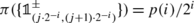.

We let $$\mathcal{D}$$ be uniform on $$\mathcal{X}= [0,1]$$. Then for each i∈ℕ s.t. p(i)≥q(i), there is a p(i) probability the target interval has width 2i, and given this any algorithm requires ∝2i expected number of requests to determine which of these 2i intervals is the target, failing which the error rate is at least 2i. In particular, letting ε i =p(i)/2i+1, any correct algorithm has sample complexity at least ∝p(i)⋅2i for ε=ε i . Noting p(i)⋅2iq(i)⋅2ig(q(i)/2i+1)≥g(ε i ), this implies there exist arbitrarily small values of ε>0 for which the optimal sample complexity is at least ∝g(ε), so that the sample complexity is not o(g(ε)).

For any s(ε)=o(1/ε), there exists a monotonic g(ε)=o(1/ε) such that s(ε)=o(g(ε)). Thus, constructing π as above for this g, we have that the sample complexity is not o(g(ε)), and therefore not O(s(ε)). So at least for the space of interval classifiers, the specific o(1/ε) asymptotic dependence on ε is inherently π-dependent. This argument also illustrates that the o(1/ε) result in Theorem 4 is essentially the strongest possible at this level of generality (i.e., without saying more about ℂ, $$\mathcal{D}$$, or π).

## Conclusions

We have shown that when learning a sequence of i.i.d. target concepts from a known VC class, with an unknown distribution from a known totally bounded family, transfer learning can lead to amortized average sample complexity close to that achievable by an algorithm with direct knowledge of the targets’ distribution. Furthermore, for the problem of active learning (with self-verification), we have shown that this latter quantity is always o(1/ε), where ε is the desired expected error rate. This represents an improvement in the asymptotic dependence on ε compared to the general results provable for active learning without transfer.

1. 1.

Furthermore, it is not difficult to see that we can take this $$\mathcal{E}$$ to be measurable in the h argument.

## References

1. Ando, R. K., & Zhang, T. (2004). A framework for learning predictive structures from multiple tasks and unlabeled data (Technical Report RC23462). IBM T.J. Watson Research Center.

2. Ando, R. K., & Zhang, T. (2005). A framework for learning predictive structures from multiple tasks and unlabeled data. Journal of Machine Learning Research, 6, 1817–1853.

3. Balcan, M.-F., Broder, A., & Zhang, T. (2007). Margin based active learning. In Proceedings of the 20th conference on learning theory.

4. Balcan, M.-F., Beygelzimer, A., & Langford, J. (2009). Agnostic active learning. Journal of Computer and System Sciences, 75(1), 78–89.

5. Balcan, M.-F., Hanneke, S., & Wortman Vaughan, J. (2010). The true sample complexity of active learning. Machine Learning, 80(2–3), 111–139.

6. Baram, Y., El-Yaniv, R., & Luz, K. (2004). Online choice of active learning algorithms. The Journal of Machine Learning Research, 5, 255–291.

7. Baxter, J. (1997). A Bayesian/information theoretic model of learning to learn via multiple task sampling. Machine Learning, 28, 7–39.

8. Baxter, J. (2000). A model of inductive bias learning. The Journal of Artificial Intelligence Research, 12, 149–198.

9. Ben-David, S., & Schuller, R. (2003). Exploiting task relatedness for multiple task learning. In Conference on learning theory.

10. Beygelzimer, A., Dasgupta, S., & Langford, J. (2009). Importance weighted active learning. In Proceedings of the international conference on machine learning.

11. Campbell, C., Cristianini, N., & Smola, A. (2000). Query learning with large margin classifiers. In International conference on machine learning.

12. Carbonell, J. G. (1983). Learning by analogy: formulating and generalizing plans from past experience. In R. S. Michalski, J. G. Carbonell, & T. M. Mitchell (Eds.), Machine learning, an artificial intelligence approach. Palo Alto: Tioga Press.

13. Carbonell, J. G. (1986). Derivational analogy: a theory of reconstructive problem solving and expertise acquisition. In R. S. Michalski, J. G. Carbonell, & T. M. Mitchell (Eds.), Machine learning, an artificial intelligence approach, Vol. II. San Mateo: Morgan Kaufmann.

14. Caruana, R. (1997). Multitask learning. Machine Learning, 28, 41–75.

15. Castro, R., & Nowak, R. (2008). Minimax bounds for active learning. IEEE Transactions on Information Theory, 54(5), 2339–2353.

16. Cohn, D., Atlas, L., & Ladner, R. (1994). Improving generalization with active learning. Machine Learning, 15(2), 201–221.

17. Dasgupta, S. (2004). Analysis of a greedy active learning strategy. In Advances in neural information processing systems (pp. 337–344). Cambridge: MIT Press.

18. Dasgupta, S. (2005). Coarse sample complexity bounds for active learning. In Proc. of neural information processing systems (NIPS).

19. Dasgupta, S., Hsu, D., & Monteleoni, C. (2008). A general agnostic active learning algorithm. In Advances in neural information processing systems (Vol. 20).

20. Dasgupta, S., Kalai, A. T., & Monteleoni, C. (2009). Analysis of perceptron-based active learning. Journal of Machine Learning Research, 10, 281–299.

21. Devroye, L., & Lugosi, G. (2001). Combinatorial methods in density estimation. New York: Springer.

22. Donmez, P., & Carbonell, J. (2008). Paired sampling in density-sensitive active learning. In Proceedings of the 10th international symposium on artificial intelligence and mathematics (SIAM).

23. Donmez, P., Carbonell, J., & Bennett, P. (2007). Dual strategy active learning. In Proceedings of the 18th European conference on machine learning.

24. Evgeniou, T., & Pontil, M. (2004). Regularized multi-task learning. In ACM SIGKDD conference on knowledge discovery and data mining.

25. Evgeniou, T., Micchelli, C., & Pontil, M. (2005). Learning multiple tasks with kernel methods. Journal of Machine Learning Research, 6, 615–637.

26. Freund, Y., Seung, H. S., Shamir, E., & Tishby, N. (1997). Selective sampling using the query by committee algorithm. In Machine learning (pp. 133–168).

27. Friedman, E. (2009). Active learning for smooth problems. In Proceedings of the 22nd conference on learning theory.

28. Hanneke, S. (2007a). A bound on the label complexity of agnostic active learning. In Proc. of the 24th international conference on machine learning.

29. Hanneke, S. (2007b). Teaching dimension and the complexity of active learning. In Proc. of the 20th annual conference on learning theory (COLT).

30. Hanneke, S. (2009). Theoretical foundations of active learning. Ph.D. thesis, Machine Learning Department, School of Computer Science, Carnegie Mellon University.

31. Hanneke, S. (2011). Rates of convergence in active learning. Annals of Statistics, 39(1), 333–361.

32. Haussler, D., Kearns, M., & Schapire, R. (1992). Bounds on the sample complexity of Bayesian learning using information theory and the VC dimension. In Machine learning (pp. 61–74). San Mateo: Morgan Kaufmann.

33. He, J., & Carbonell, J. (2008). Rare class discovery based on active learning. In Proceedings of the 10th international symposium on artificial intelligence and mathematics.

34. Kääriäinen, M. (2006). Active learning in the non-realizable case. In Proc. of the 17th international conference on algorithmic learning theory.

35. Kallenberg, O. (2002). Foundations of modern probability (2nd ed.). New York: Springer.

36. Kolodner, J. (Ed.) (1993). Case-based learning. Dordrecht: Kluwer Academic.

37. Koltchinskii, V. (2010). Rademacher complexities and bounding the excess risk in active learning. Journal of Machine Learning Research, 11, 2457–2485.

38. McCallum, A., & Nigam, K. (1998). Employing EM and pool-based active learning for text classification. In International conference on machine learning.

39. Micchelli, C., & Pontil, M. (2004). Kernels for multi-task learning. In Advances in neural information processing (Vol. 18).

40. Nguyen, H., & Smeulders, A. (2004). Active learning using pre-clustering. In International conference on machine learning.

41. Nowak, R. D. (2008). Generalized binary search. In Proceedings of the 46th annual Allerton conference on communication, control, and computing.

42. Schervish, M. J. (1995). Theory of statistics. New York: Springer.

43. Silver, D. L. (2000). Selective transfer of neural network task knowledge. Ph.D. thesis, Computer Science, University of Western Ontario.

44. Thrun, S. (1996). Is learning the n-th thing any easier than learning the first? In Advances in neural information processing systems (Vol. 8).

45. Tong, S., & Koller, D. (2001). Support vector machine active learning with applications to text classification. Journal of Machine Learning Research, 2(1), 45–66.

46. Vapnik, V. (1982). Estimation of dependencies based on empirical data. New York: Springer.

47. Veloso, M. M., & Carbonell, J. G. (1993). Derivational analogy in prodigy: automating case acquisition, storage and utilization. Machine Learning, 10, 249–278.

48. Wang, L. (2009). Sufficient conditions for agnostic active learnable. In Advances in neural information processing systems (Vol. 22).

49. Yatracos, Y. G. (1985). Rates of convergence of minimum distance estimators and Kolmogorov’s entropy. Annals of Statistics, 13, 768–774.

Download references

## Acknowledgements

We would like to extend our sincere thanks to Avrim Blum for several thought-provoking discussions on this topic.

## Author information

Authors

### Corresponding author

Correspondence to Liu Yang.

## Additional information

Editor: Tong Zhang.

## Rights and permissions

Reprints and Permissions

## About this article

### Cite this article

Yang, L., Hanneke, S. & Carbonell, J. A theory of transfer learning with applications to active learning. Mach Learn 90, 161–189 (2013). https://doi.org/10.1007/s10994-012-5310-y

Download citation

• Received:

• Revised:

• Accepted:

• Published:

• Issue Date:

### Keywords

• Transfer learning
• Multi-task learning
• Active learning
• Statistical learning theory
• Bayesian learning
• Sample complexity